# Twin Primes Conjecture / Proof via Prime Sieve Method

An almost proof of the The Twin Primes Conjecture...SiteMap of SciRealm | About John | John's Resume/C.V./Bio | Send email to John John's Science, Math, Philosophy Stuff: The Science Realm: John's Virtual Sci-Tech Universe | 4-Vectors | Ambigrams | Antipodes | Covert Ops Fragments | Cyrillic Projector | Forced Induction (Sums Of Powers Of Integers) | Fractal Tree | Frontiers | IFS Fractals (Assembly) | IFS Fractals (Javascript) | JavaScript Graphics | Kid Science | Kryptos | Philosophy | Photography | Physics-Planck Constant Measurement Via LEDs | Prime Sieve | QM from SR | QM from SR-Simple RoadMap | QM from SR-Simple RoadMap (PDF) | SR 4-Vector & Tensor Calculator |Quantum Phase | Quotes | RuneQuest Cipher Challenge | Secret Codes & Ciphers | Scientific Calculator (complex capable) | Science+Math | Sci-Pan Pantheist Poems | Stereograms | SuperMagicSqr4x4 |Turkish Grammar |Melike Wilson Art Site last modified: 2021-Aug Welcome to Relativistic Quantum Reality: Virtual worlds of imaginary particles: The dreams stuff is made of: Life, the eternal ghost in the machine... This site is dedicated to the quest for knowledge and wisdom, through science, mathematics, philosophy, invention, and technology.  May the treasures buried herein spark the fires of imagination like the twinkling jewels of celestial light crowning the midnight sky... Quantum Mechanics is derivable from Special Relativity See SRQM - QM from SR - Simple RoadMap (.html) See SRQM - QM from SR - Simple RoadMap (.pdf) See SRQM - Online SR 4-Vector & Tensor Calculator See Online Complex-capable RPN Scientific Calculator ***Learn, Discover, Explore***Note: This work is not finished, but if you notice errors or have comments, please let me know.
Twin Primes Conjecture / Proof via Prime Sieve Method

The Prime Sieve is a constructive method or algorithm for finding prime numbers. This document will analyze the method in some detail, hopefully adding to our mathematical knowledge.

Summary of Twin Primes Conjecture Proof.
A sieve method is used to find the mathematical form of various prime numbers.
These mathematical forms split the natural #'s into various branches.
Within a given branch, an assured prime range can be established.
These assured prime ranges form an overlapping sequence by construction.
It was proved that there are an infinite number prime #'s on each branch.
The branches can be further pared to leave only the twin primes.
It is shown that the such paring cannot cut all the branches off.
Since there were an infinite # of primes on each branch, there are an infinite # of Twin Primes.

Now, we will examine the related procedure for twin primes:

Sieve-N:
Finds primes>N
The repeat distance is Prime*Prime*Prime*...*N=2*3*5*7*11*...*N=Primorial[N]
Possible forms is LastForms*(N-1)
Includes: All primes>N and composites with prime factors>N
Excludes: All multiples of N  not already excluded, (LastForms) branches

1st failure at (NextPrime[N])2
2nd failure at (NextPrime[N]*NextPrime[NextPrime[N]])
Assured prime range: N < primes < (NextPrime[N]*NextPrime[NextPrime[N]])
Mathematical Form is [RepeatDistance*nN+LastRepeat*cN+k] where possible cN values are from 0..(N-1)

Apply PrimeSieve-1:
Finds: primes>1
The repeat distance is: 1
Possible forms/branches is: 1
Includes: All whole numbers, which may be zero or prime or composite
Excludes: All non-whole numbers, i.e. fractions, irrationals, etc.
1st failure at: 4=2*2=22
2nd failure at: 6=2*3
Assured prime range: 1 < primes < 6
 # Mathematical Form c1 values (0..0) Old/New/Extended Primes Bad/Failed 1 1 < [n1+c1] < 22 = 4 (6) which simplifies to: 1 < [n1] < 22 = 4 (6) 0 or undefined 1,2,3+5,7? 4,6

Next confirmed prime is 2...
We need [n1] mod 2 <> 0
Try (n1=2n2+c2)
[2n2+c2] mod 2 <> 0
which simplifies to:
[c2] mod 2 <> 0
Solution is Complement of (0..1), c2=1

Apply PrimeSieve-2:
Finds: primes>2
The repeat distance is: 2
Possible forms/branches is: 1*(2-1)=1
Includes: All primes>2 and composites with prime factors>2
Excludes: All multiples of 2, [2n2+0]
1st failure at: 9=3*3=32
2nd failure at: 15=3*5
Assured prime range: 2 < primes < 15

Notes: At this point, all primes of this mathematical form are potentially twin, as each is spaced 2 apart from its nearest neighbor.

 # Mathematical Form c2 values (0..1) Old/New/Extended Primes Bad/Failed 1 2 < [2n2+c2] < 32 = 9 (15) which simplifies to: 2 < [2n2+1] < 32 = 9 (15) Complement =1 x, 1,(3+5+7),,(11+13),,(17?+19?),... 9,15

Next confirmed prime is 3...
We need [2n2+1] mod 3 <> 0
Try (n2=3n3+c3)
[6n3+2c3+1] mod 3 <> 0
which simplifies to:
[2c3+1] mod 3 <> 0
Solution is Complement of (0..2), c3=0,2

Apply PrimeSieve-3:
Finds: TwinPrime pairs>3
The repeat distance is: 2*3=6
Possible forms/branches is: 1*(3-1)=2
Possible twin branches is: 1*(3-2)=1
Includes: All primes>3 and composites with prime factors>3
Excludes: All multiples of 3 not already excluded, i.e. [6n3+3]
1st failure at: 25=5*5=52
2nd failure at: 35=5*7
Assured prime range: 3 < primes < 35

 # Mathematical Form c3 values (0..2) Old/New/Extended Primes Bad/Failed 1 3 < [6n3+2c3+1] < 52 = 25 (35) which simplifies to: 3 < [6n3+1] < 52 = 25 (35) & 3 < [6n3+5] < 52 = 25 (35) Complement =0,2 1,7,13,19,,31 x, -1,5,11,17,23,29 25 35

Notes:
This can be rewritten to a more convenient form for twin primes, since (5) mod 3 = (-1) mod 3
At this point, the twin prime structure has become apparent. We can combine the two above branches into a single twin-prime branch with the notation.
We do not lose any info, we are just shifting the start point of one of the branches for convenience.
 # Mathematical Form c3 values (0..2) Old/New/Extended Primes 1 3 < [6n3+2c3+1] < 52 = 25 (35) which simplifies to: 3 < [6n3�1] < 52 = 25 (35) Complement =0,2 (-1,1),(5+7),(11+13),(17+19),(23,25),(29+31),(35,37?),...

Next confirmed prime is 5...
We need [6n3�1] mod 5 <> 0
Try (n3=5n5+c5)

 [30n5+6c5+1] mod 5 <> 0 which simplifies to: [c5+1] mod 5 <> 0 Solution is Complement of (0..4) c5=0,1,2,3 [30n5+6c5-1] mod 5 <> 0 which simplifies to: [c5-1] mod 5 <> 0 Solution is Complement of (0..4) c5=0,2,3,4

The solutions are slightly different from the regular prime sieve since I took the starting points from  ([6n3+1] & [6n3+5] ) to ( [6n3+1] & [6n3-1] ). If you plug in the allowed values, you will see that you get the same number sequences.

There is one more thing that makes the twin prime sieve different from the regular prime sieve.  Before, each branch had to survive the modulus operation individually.
Now, BOTH branches must obey BOTH constraints; otherwise, you would get pairing of a prime with a composite.

Therefore, we have [30n5+6c5�1] mod 5 <> 0, with the solution of:
c5=Complement[1,4] of (0..4)
c5=0,2,3

Thus, we have excluded the following branches:
Composites of 5 in branch [30n5+6c5+1]
Composites of 5 in branch [30n5+6c5-1]
Prime partners in branch [30n5+6c5+1] of a composite partner in branch [30n5+6c5-1]
Prime partners in branch [30n5+6c5-1] of a composite partner in branch [30n5+6c5+1]
Hence, only TwinPrimes remain within the defined range of the Twin Sieve.

Apply TwinSieve-5:
Finds: TwinPrime pairs>5
The repeat distance is: 2*3*5=30
Possible twin branches branches is: 1*(5-2)=3
Includes: All TwinPrimes>5 and composites with prime factors>5
Excludes: All multiples of 5 not already excluded, and prime partners of composites in the twin branch
1st failure at: 49=7*7=72
2nd failure at: 77=7*11
Assured TwinPrime range: 5 < primes < 77

 # Mathematical Form c5 values (0..4) Old/New/Extended Primes 1 5 < [30n5+6c5+0�1] < 72 = 49 (77) Complement[1,4] =0,2,3 (-1,1),(29+31),(59+61),(89?,91),... (11+13),(41+43),(71+73),(101?+103?)... (17+19),(47,49),77

Next confirmed prime is 7...
We need [30n5+6c5�1] mod 7 <> 0 for c5=0,2,3
Try (n5=7n7+c7)
[210n7+30c7+6c5�1] mod 7 <> 0 for c5=0,2,3

 [210n7+30c7+0�1] mod 7 <> 0 which simplifies to: [2c7�1] mod 7 <> 0 Solution is Complement[3,4] of (0..6) c7=0,1,2,5,6 [210n7+30c7+12�1] mod 7 <> 0 which simplifies to: [2c7+5�1] mod 7 <> 0 Solution is Complement[4,5] of (0..6) c7=0,1,2,3,6 [210n7+30c7+18�1] mod 7 <> 0 which simplifies to: [2c7+4�1] mod 7 <> 0 Solution is Complement[1,2] of (0..6) c7=0,3,4,5,6

Apply TwinSieve-7:
Finds: TwinPrime pairs>7
The repeat distance is: 2*3*5*7=210
Possible twin branches branches is: 3*(7-2)=15
Includes: All TwinPrimes>7 and composites with prime factors>7
Excludes: All multiples of 7 not already excluded, and prime partners of composites in the twin branch
1st failure at: 121=11*11=112
2nd failure at: 143=11*13
Assured TwinPrime range: 7 < primes < 153

 # Mathematical Form c7 values (0..6) Old/New/Extended Primes 1 7 < [210n7+30c7+0�1] < 112 = 121 (143) Complement[3,4] of (0..6) c7=0,1,2,5,6 (-1,1),(209?,211?),... (29+31),(239?,241?),... (59+61),(269?,271?),... x, x, (149?,151?),... (179?,181?),... 2 7 < [210n7+30c7+12�1] < 112 = 121 (143) Complement[4,5] of (0..6) c7=0,1,2,3,6 (11+13),(221?,223?),... (41+43),(251?,253?),... (71+73),(281?,283?),... (101+103),(311?,313?),... x, x, (191?+193?),... 3 7 < [210n7+30c7+18�1] < 112 = 121 (143) Complement[1,2] of (0..6) c7=0,3,4,5,6 (17+19),(227?,229?),... x, x, (107+109),(217?,219?) (137+139),(247?,249?) (167?,169?),... (197?,199?),...

Next confirmed prime is 11...

We need [210n7+30c7+k�1] mod 11 <> 0 for k=0,12,18 and c7=various
Try (n7=11n11+c11)
[2310n11+210c11+30c7+k�1] mod 11 <>0

Apply TwinSieve-11:
Finds: TwinPrime pairs>11
The repeat distance is: 2*3*5*7*11=2310
Possible twin branches branches is: 15*(11-2)=135
Includes: All TwinPrimes>11 and composites with prime factors>11
Excludes: All multiples of 11 not already excluded, and prime partners of composites in the twin branch
1st failure at: 169=13*13=132
2nd failure at: 221=13*17
Assured TwinPrime range: 11 < primes < 221

 # Mathematical Form c11 values (0..10) Old/New/Extended Primes 1 11 < [2310n11+210c11+0�1] < 132 = 169 (221) c11=Complement[1,10] of (0..10) (-1,1),x,... 2 11 < [2310n11+210c11+12�1] < 132 = 169 (221) c11=Complement[0,9] of (0..10) x,(221,223)... 3 11 < [2310n11+210c11+18�1] < 132 = 169 (221) c11=Complement[3,5] of (0..10) (17+19),... 4 11 < [2310n11+210c11+30�1] < 132 = 169 (221) c11=Complement[2,4] of (0..10) (29+31),... 5 11 < [2310n11+210c11+42�1] < 132 = 169 (221) c11=Complement[1,3] of (0..10) (41+43),x,... 6 11 < [2310n11+210c11+60�1] < 132 = 169 (221) c11=Complement[5,7] of (0..10) (59+61),... 7 11 < [2310n11+210c11+72�1] < 132 = 169 (221) c11=Complement[4,6] of (0..10) (71+73),... 8 11 < [2310n11+210c11+102�1] < 132 = 169 (221) c11=Complement[7,9] of (0..10) (101+103),... 9 11 < [2310n11+210c11+108�1] < 132 = 169 (221) c11=Complement[1,3] of (0..10) (107+109),x,... 10 11 < [2310n11+210c11+138�1] < 132 = 169 (221) c11=Complement[4,6] of (0..10) (137+139),... 11 11 < [2310n11+210c11+150�1] < 132 = 169 (221) c11=Complement[3,5] of (0..10) (149+151),... 12 11 < [2310n11+210c11+168�1] < 132 = 169 (221) c11=Complement[7,9] of (0..10) (167,169),... 13 11 < [2310n11+210c11+180�1] < 132 = 169 (221) c11=Complement[6,8] of (0..10) (179+181),... 14 11 < [2310n11+210c11+192�1] < 132 = 169 (221) c11=Complement[5,7] of (0..10) (191+193),... 15 11 < [2310n11+210c11+198�1] < 132 = 169 (221) c11=Complement[1,10] of (0..10) (197+199),x,...

Next confirmed prime is 13...

We need [2310n11+210c11+k�1] mod 13 <> 0 for k=various and c7=various
Try (n11=13n13+c13)
[30030n11+2310c11+k�1] mod 13 <>0

Apply TwinSieve-13:
Finds: TwinPrime pairs>13
The repeat distance is: 2*3*5*7*11*13=30030
Possible twin branches branches is: 135*(13-2)=1485
Includes: All TwinPrimes>13 and composites with prime factors>13
Excludes: All multiples of 13 not already excluded, and prime partners of composites in the twin branch
1st failure at: 289=17*17=172
2nd failure at: 323=17*19
Assured TwinPrime range: 13 < primes < 323

 # Mathematical Form c13 values (0..12) Old/New/Extended Primes 1 13 < [30030n13+2310c13+0�1] < 172 = 289 (323) c13=Complement[] of (0..12) (-1,1),x,... 2 13 < [30030n13+2310c13+18�1] < 172 = 289 (323) c13=Complement[] of (0..12) (17+19),... 3 13 < [30030n13+2310c13+30�1] < 172 = 289 (323) c13=Complement[] of (0..12) (29+31),... 4 13 < [30030n13+2310c13+42�1] < 172 = 289 (323) c13=Complement[] of (0..12) (41+43),x,... 5 13 < [30030n13+2310c13+60�1] < 172 = 289 (323) c13=Complement[] of (0..12) (59+61),... 6 13 < [30030n13+2310c13+72�1] < 172 = 289 (323) c13=Complement[] of (0..12) (71+73),... 7 13 < [30030n13+2310c13+102�1] < 172 = 289 (323) c13=Complement[] of (0..12) (101+103),... 8 13 < [30030n13+2310c13+108�1] < 172 = 289 (323) c13=Complement[] of (0..12) (107+109),x,... 9 13 < [30030n13+2310c13+138�1] < 172 = 289 (323) c13=Complement[] of (0..12) (137+139),... 10 13 < [30030n13+2310c13+150�1] < 172 = 289 (323) c13=Complement[] of (0..12) (149+151),... 11 13 < [30030n13+2310c13+168�1] < 172 = 289 (323) c13=Complement of (0..12) x,... 12 13 < [30030n13+2310c13+180�1] < 172 = 289 (323) c13=Complement[] of (0..12) (179+181),... 13 13 < [30030n13+2310c13+192�1] < 172 = 289 (323) c13=Complement[] of (0..12) (191+193),... 14 13 < [30030n13+2310c13+198�1] < 172 = 289 (323) c13=Complement[] of (0..12) (197+199),x,... 15 13 < [30030n13+2310c13+222�1] < 172 = 289 (323) c13=Complement[] of (0..12) x,... 16 13 < [30030n13+2310c13+228�1] < 172 = 289 (323) c13=Complement[] of (0..12) (227+229),x,... 17 13 < [30030n13+2310c13+240�1] < 172 = 289 (323) c13=Complement[] of (0..12) (239+241),x,... 18 13 < [30030n13+2310c13+270�1] < 172 = 289 (323) c13=Complement[] of (0..12) (269+271),x,... 19 13 < [30030n13+2310c13+282�1] < 172 = 289 (323) c13=Complement[] of (0..12) (281+283),x,... 20 13 < [30030n13+2310c13+312�1] < 172 = 289 (323) c13=Complement[] of (0..12) (311+313),x,... 21 13 < [30030n13+2310c13+318�1] < 172 = 289 (323) c13=Complement[] of (0..12) (317+319),x,... 22 13 < [30030n13+2310c13+348�1] < 172 = 289 (323) c13=Complement[] of (0..12) (347?+349?),x,... 23 24 25

Beginning Twin Primes List:
(3+5)
(5+7)
(11+13)
(17+19)
(29+31)
(41+43)
(59+61)
(71+73)
(101+103)
(107+109)
(137+139)
(149+151)
(179+181)
(191+193)
(197+199)
(227+229)
(239+241)
(269+271)
(281+283)
(311+313)
(317+319)

Now, from the earlier Prime Sieve page, we proved that every (non-all-composite) branch has an infinite number of primes.
The Twin Prime procedure takes these SAME branches, and pares off the ones that do not contain twin primes.
There is no limit to the TwinPrime branching procedure, as before, and there are an infinite number of primes on each branch.
Therefore, there are an infinite # of TwinPrimes.

By related procedure, this also proves an infinite # of cousin primes, an infinite # of sexy primes, and so forth...

This concludes the Proof of the Twin Prime Conjecture.
JBW

* email John *
Back to the Science Realm: John's Virtual Sci+Tech Universe Email me, especially if you notice errors (which I will fix ASAP) or have interesting comments.## quantum relativity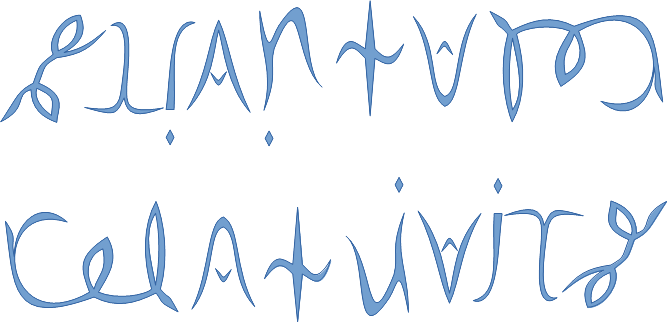See SRQM: QM from SR - The 4-Vector RoadMap (.html)
See SRQM: QM from SR - The 4-Vector RoadMap (.pdf)

SRQM Physics Diagramming Method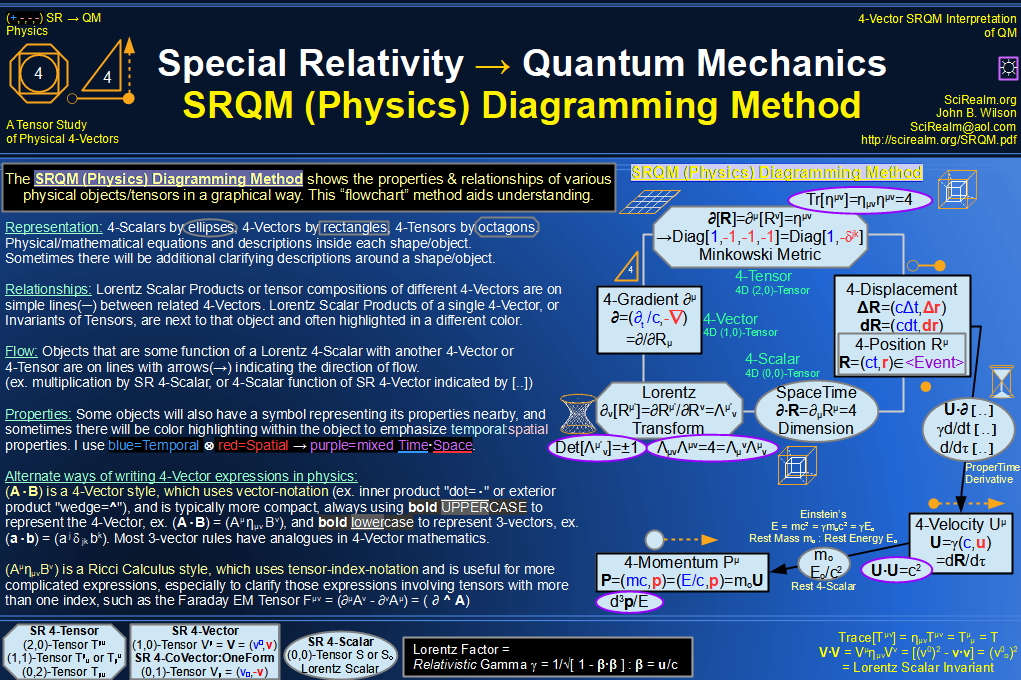SRQM 4-Vector : Four-Vector and Lorentz Scalar Diagram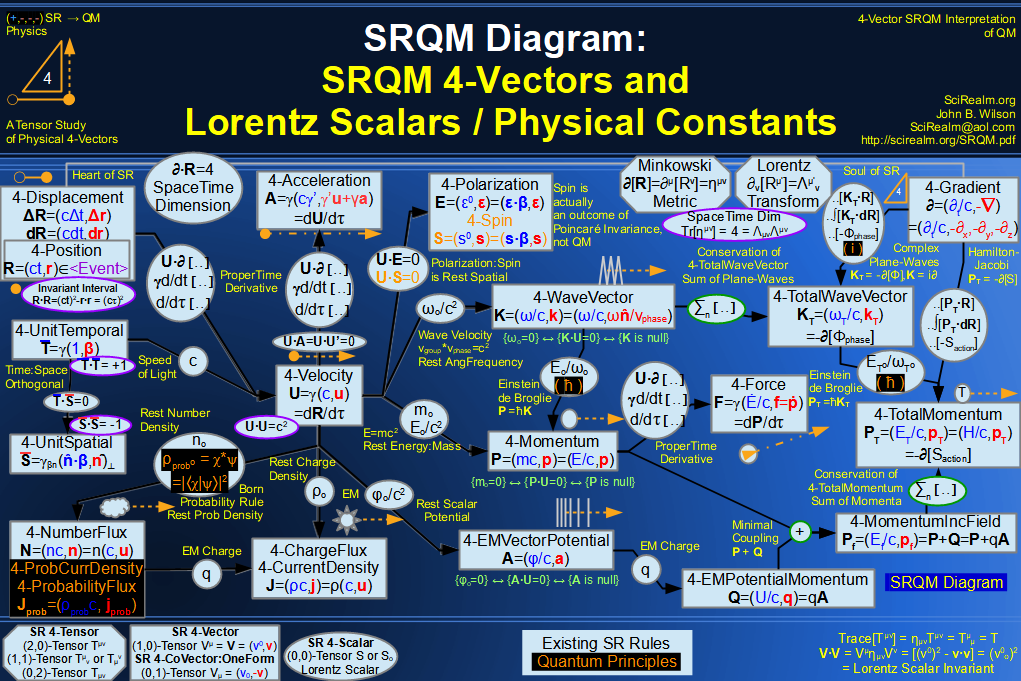SRQM + EM 4-Vector : Four-Vector and Lorentz Scalar Diagram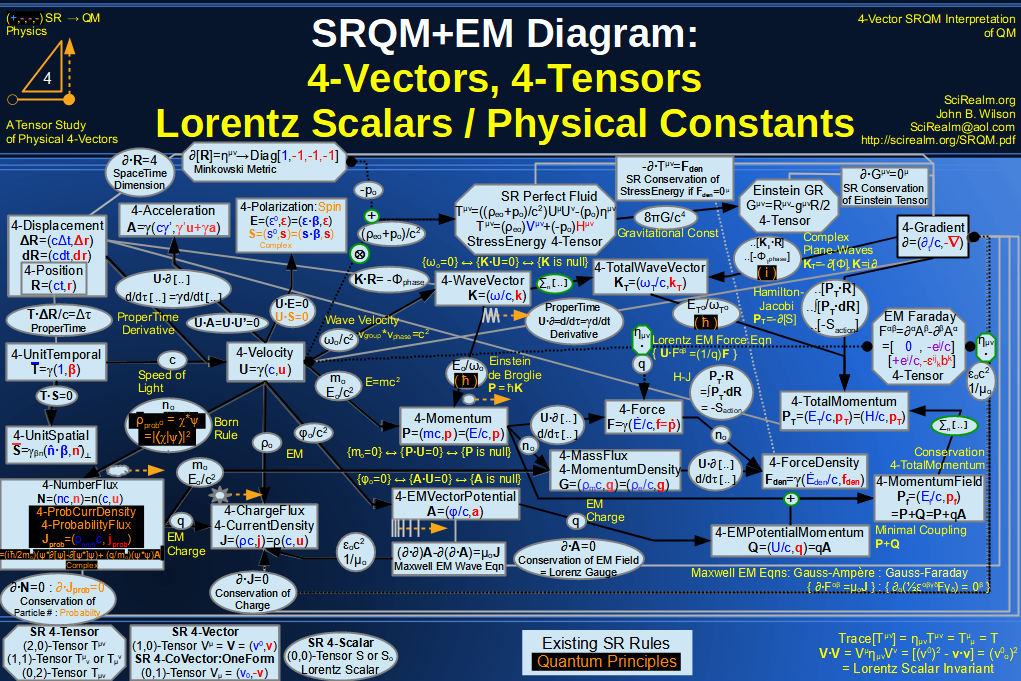SRQM + EM 4-Vector : Four-Vector and Lorentz Scalar Diagram With Tensor Invariants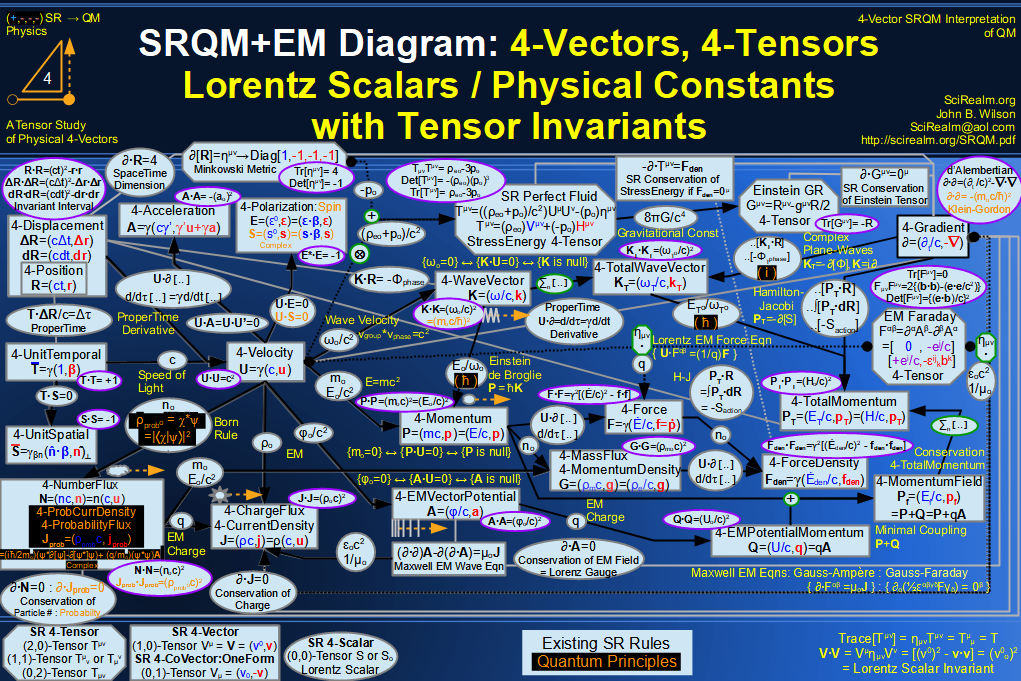SRQM 4-Vector : Four-Vector Stress-Energy & Projection Tensors Diagram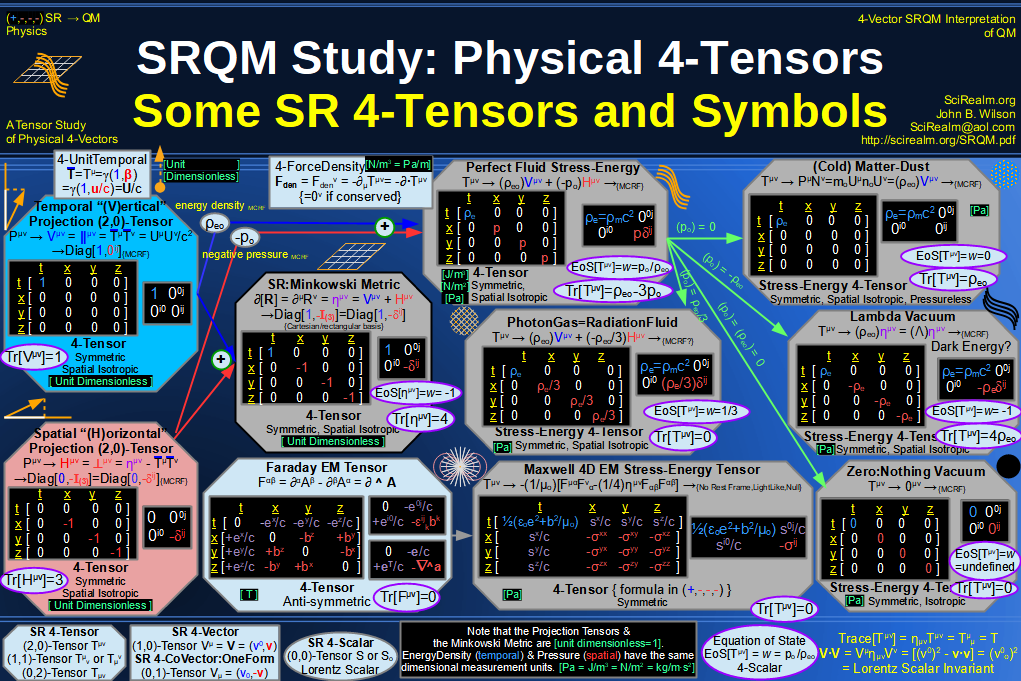SRQM 4-Vector : Four-Vector SR Quantum RoadMap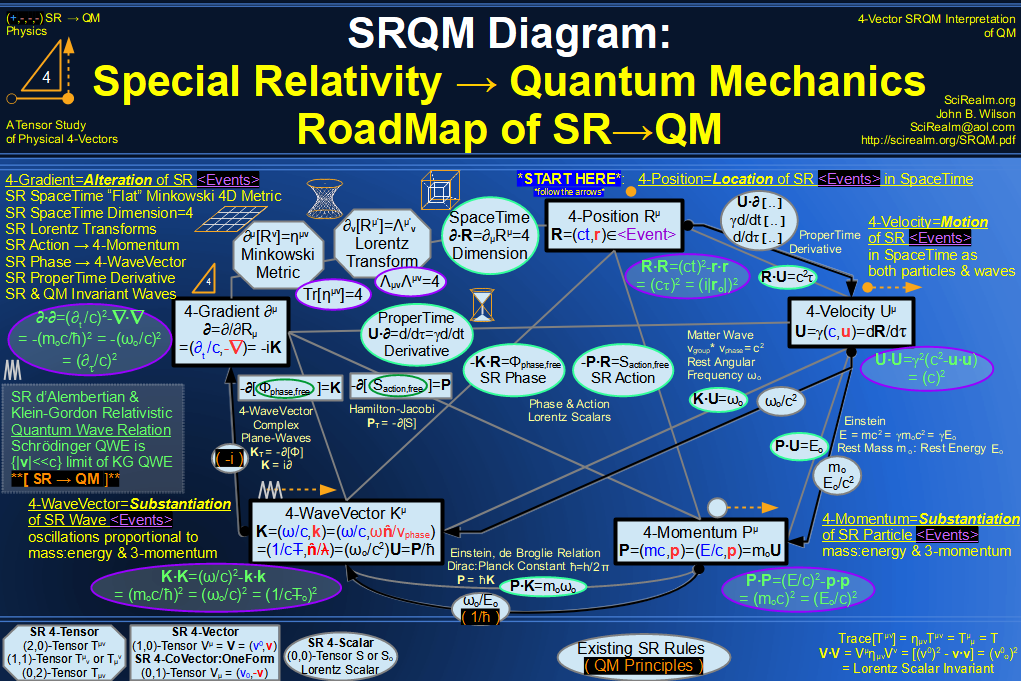SRQM + EM 4-Vector : Four-Vector SR Quantum RoadMap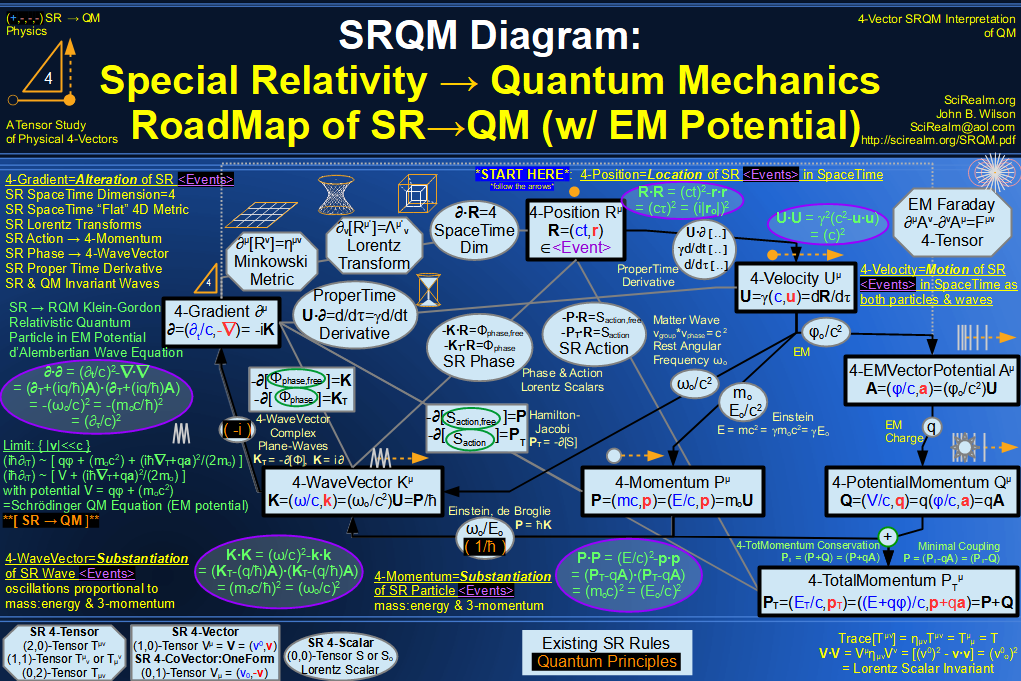SRQM + EM 4-Vector : Four-Vector SR Quantum RoadMap - Simple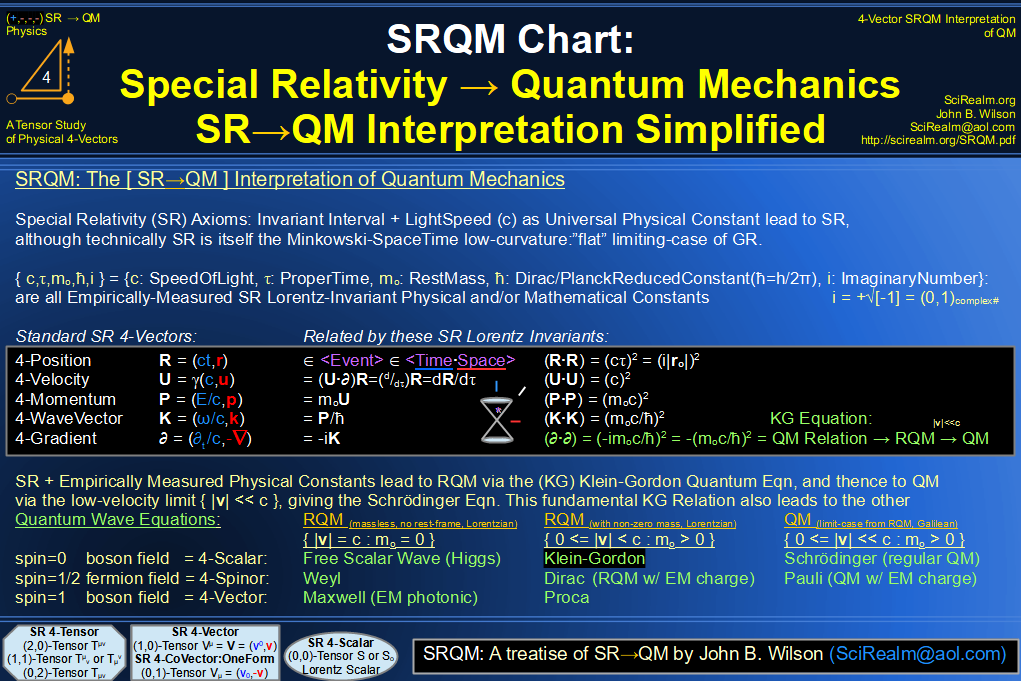SRQM 4-Vector : Four-Vector New Relativistic Quantum Paradigm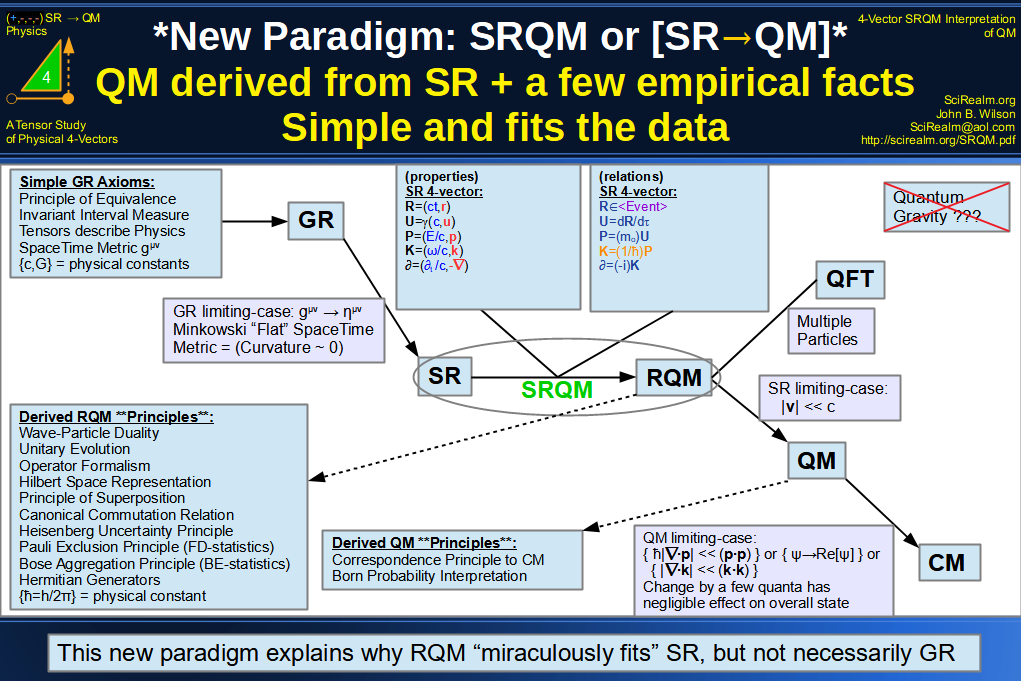SRQM + EM 4-Vector : Four-Vector New Relativistic Quantum Paradigm (with EM)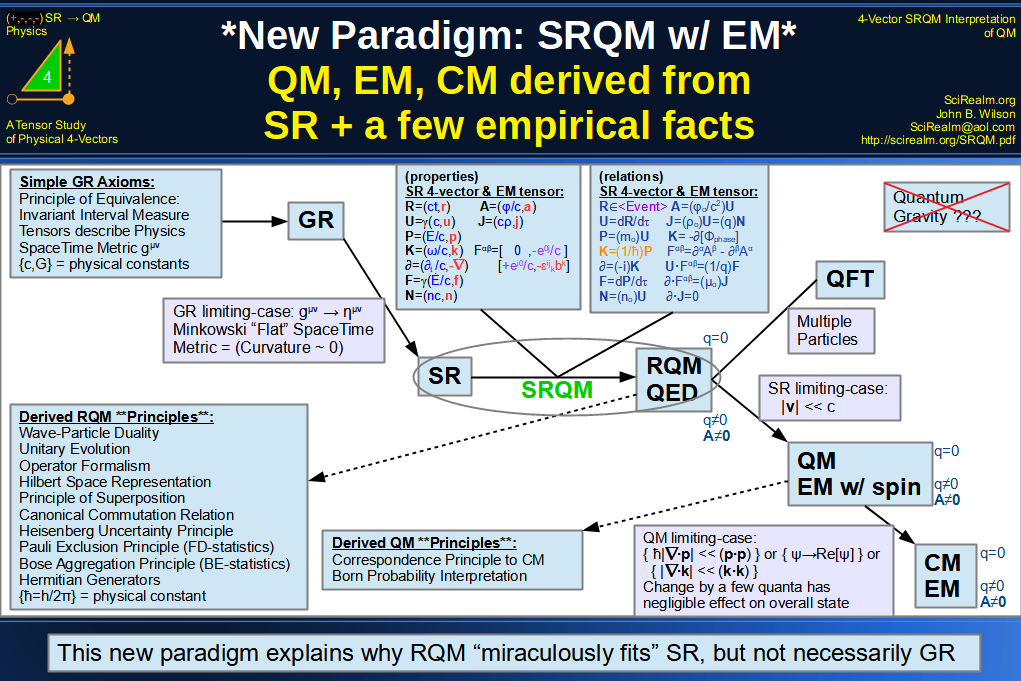SRQM 4-Vector : Four-Vector New Relativistic Quantum Paradigm - Venn Diagram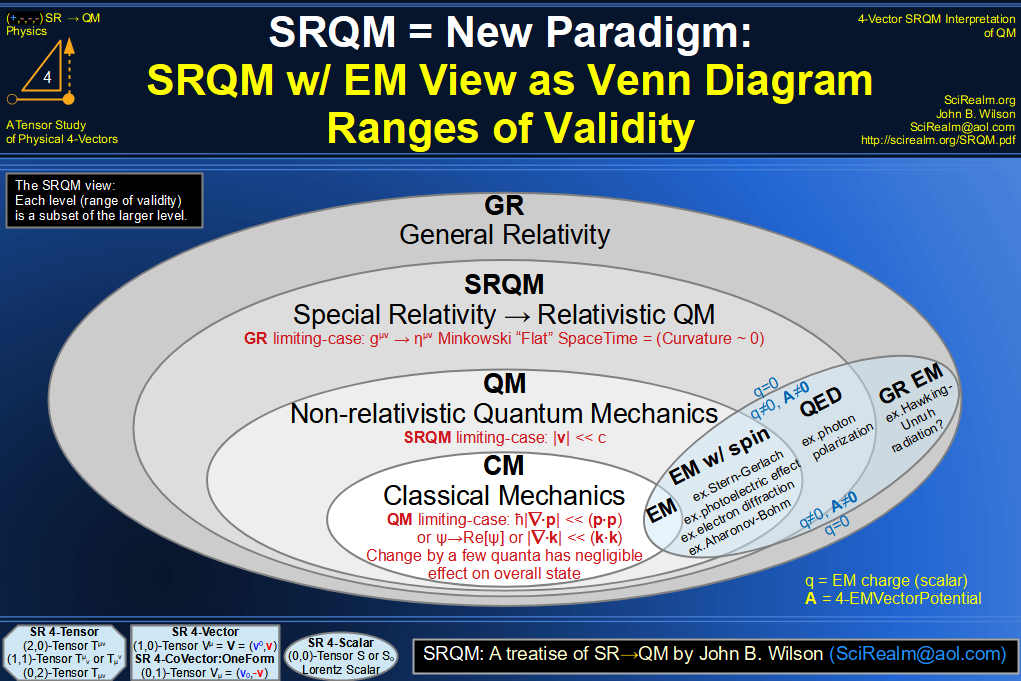SRQM 4-Vector : Four-Vector SpaceTime is 4D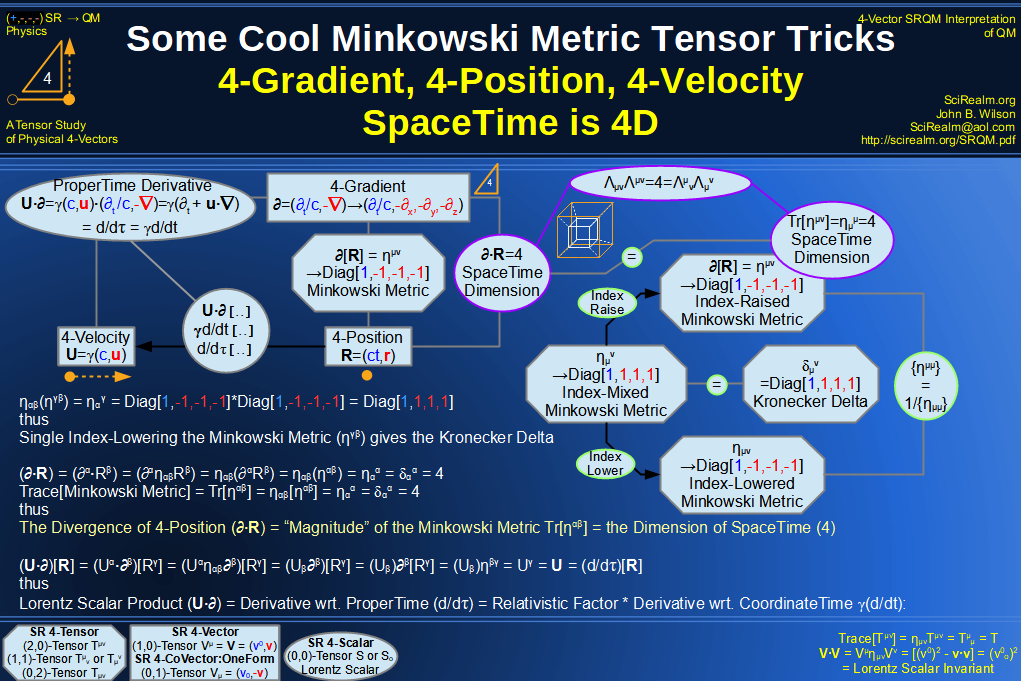SRQM 4-Vector : Four-Vector SpaceTime Orthogonality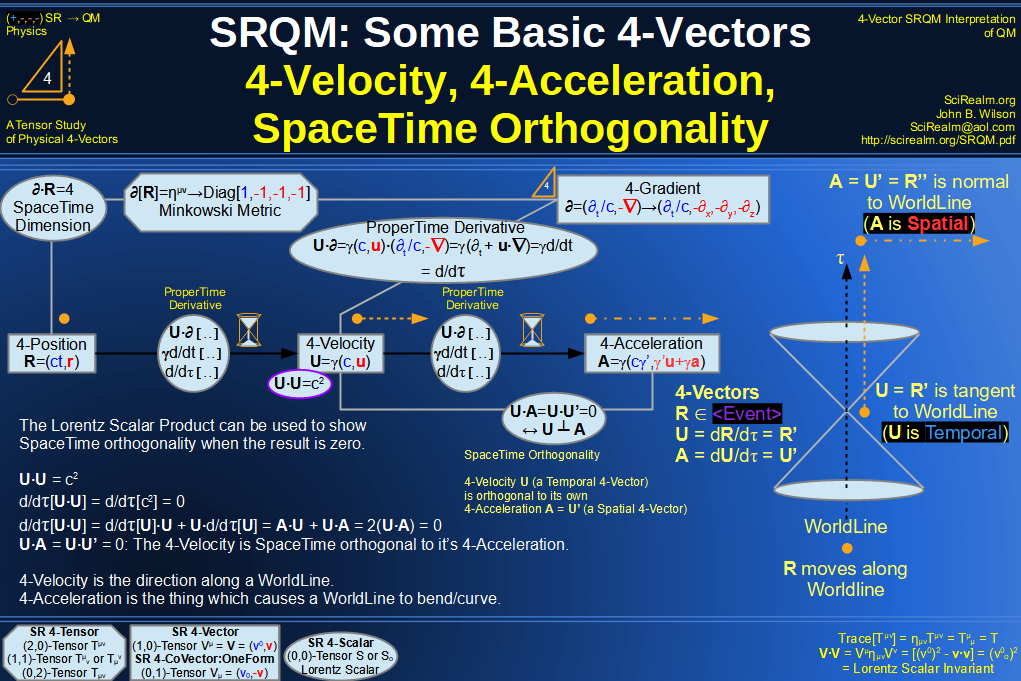SRQM 4-Vector : Four-Vector 4-Position, 4-Velocity, 4-Acceleration Diagram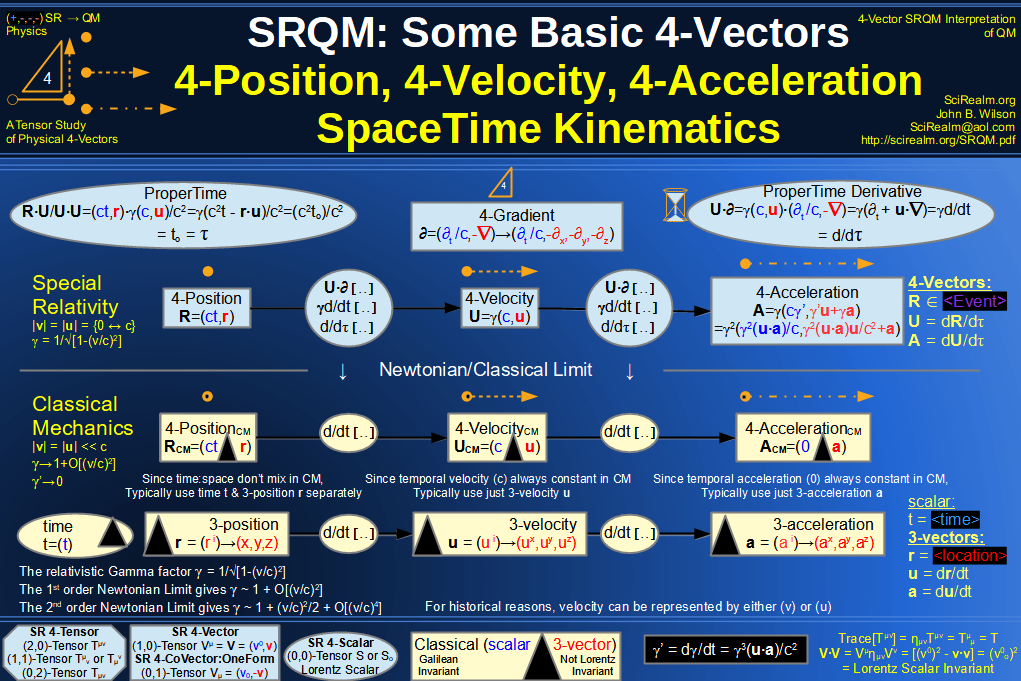SRQM 4-Vector : Four-Vector 4-Displacement, 4-Velocity, Relativity of Simultaneity Diagram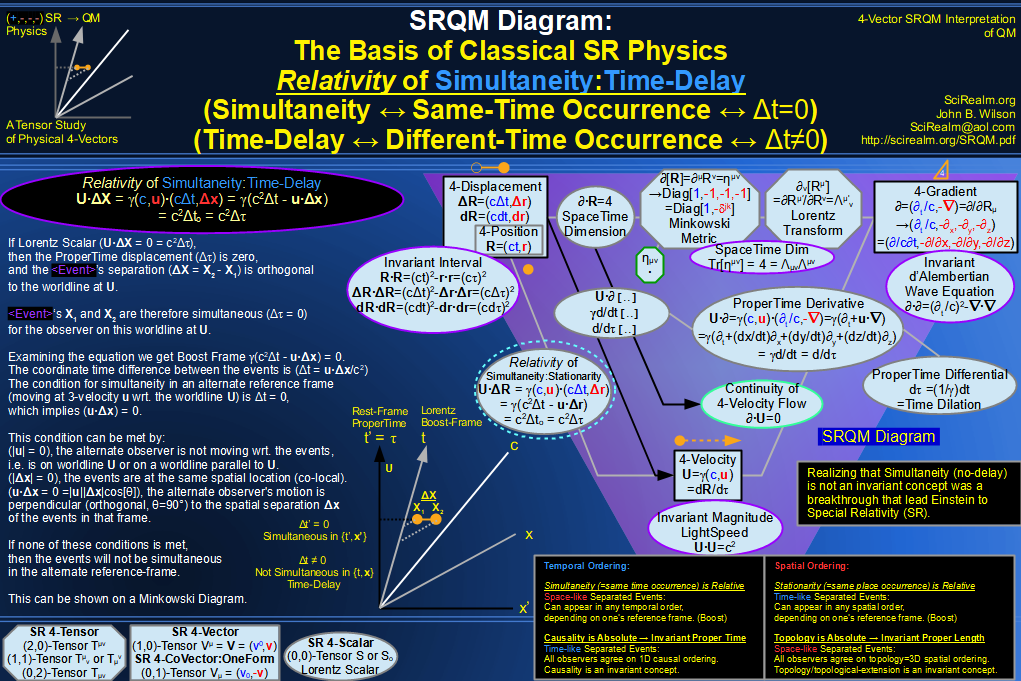SRQM 4-Vector : Four-Vector 4-Velocity, 4-Gradient, Time Dilation Diagram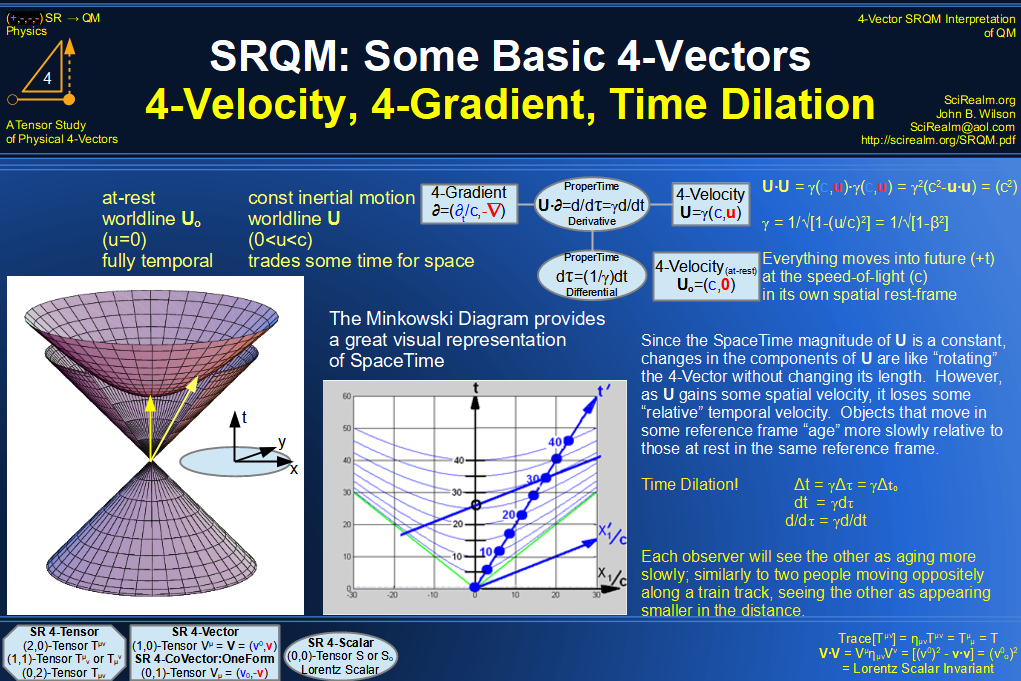SRQM 4-Vector : Four-Vector 4-Vector, 4-Velocity, 4-Momentum, E=mc^2 Diagram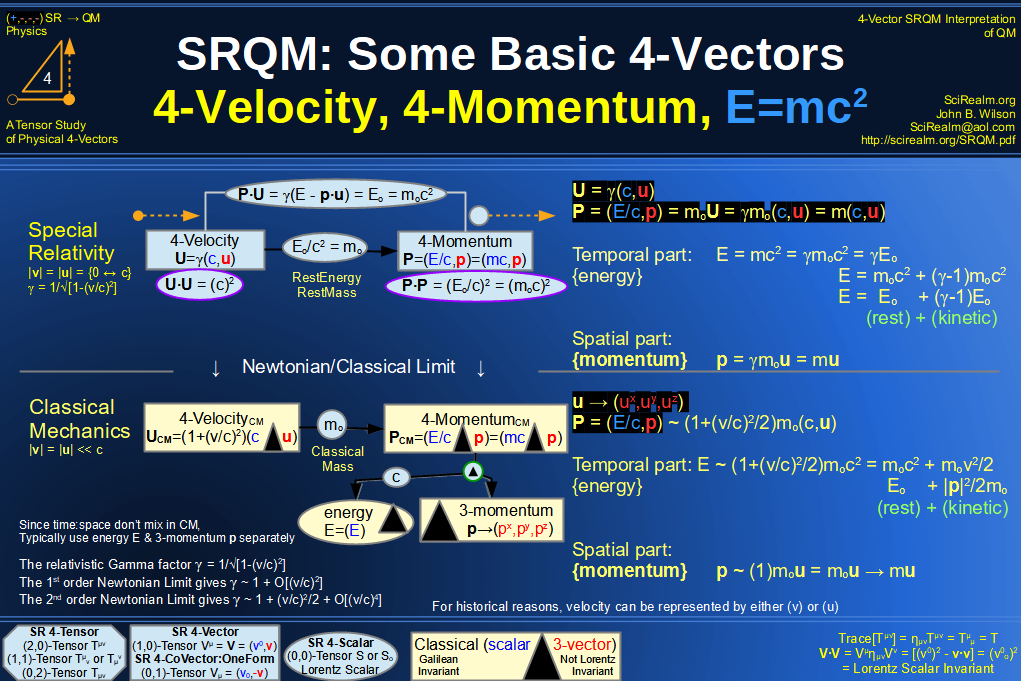SRQM 4-Vector : Four-Vector 4-Velocity, 4-WaveVector, Relativistic Doppler Effect Diagram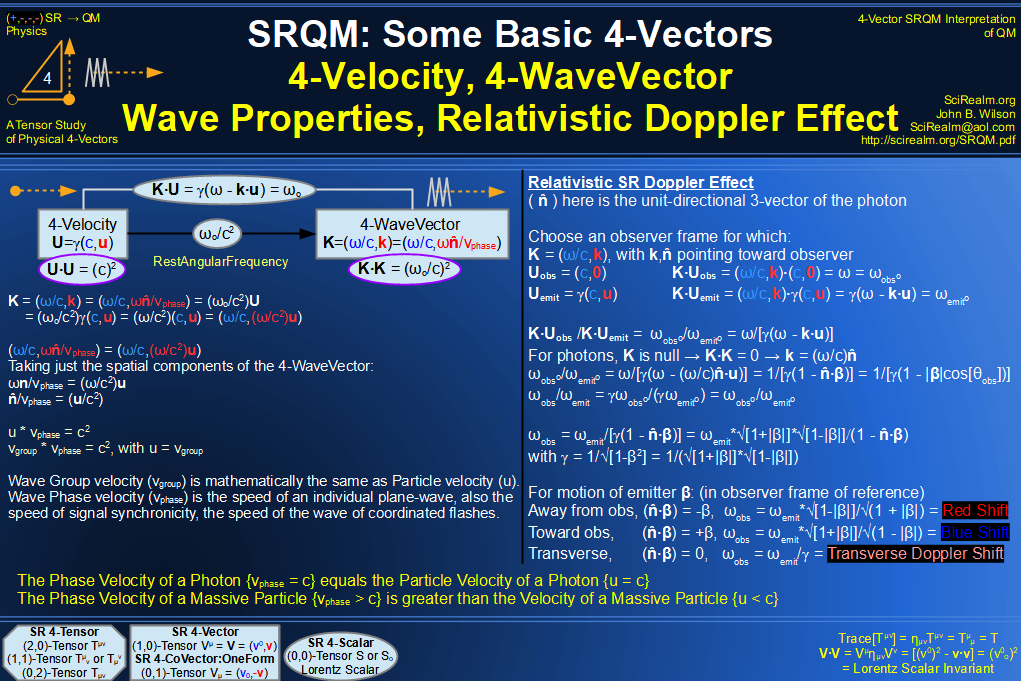SRQM 4-Vector : Four-Vector Wave-Particle Diagram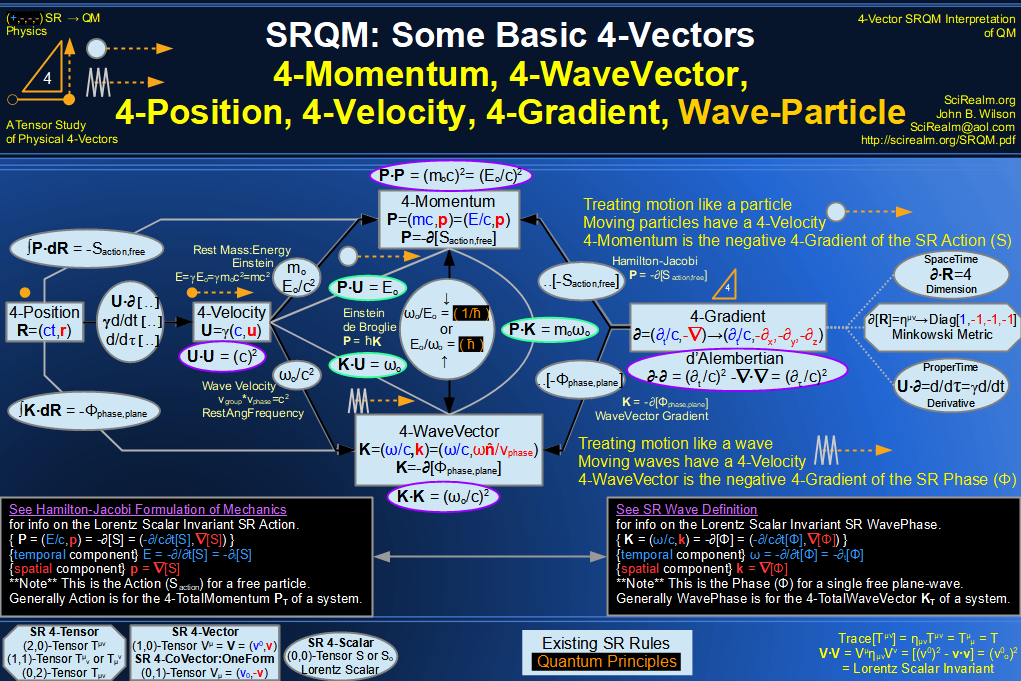SRQM 4-Vector : Four-Vector Compton Effect Diagram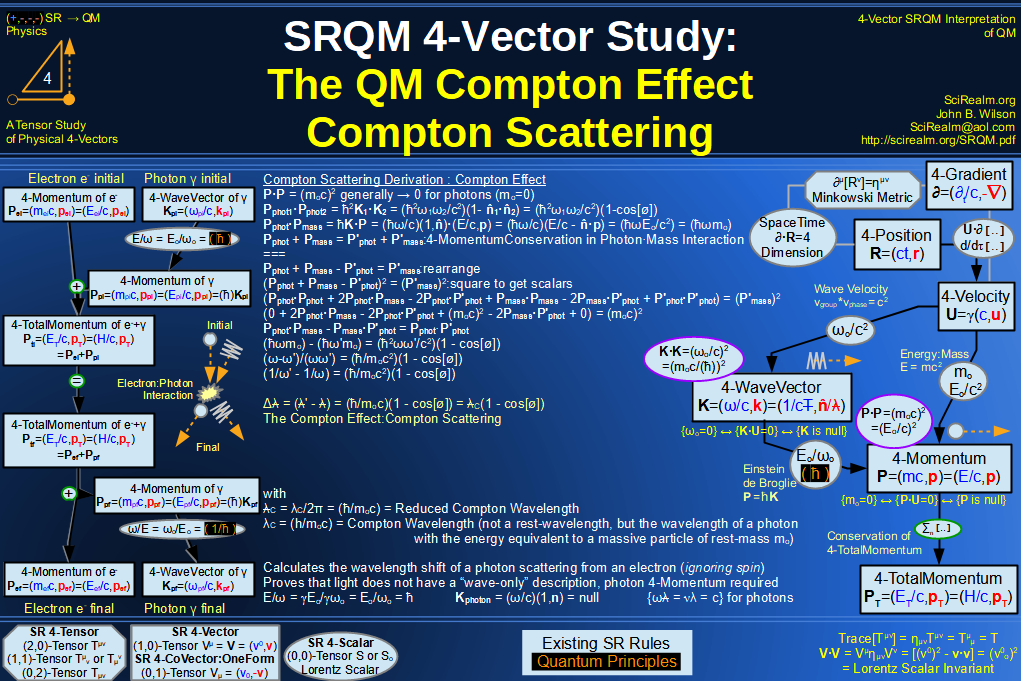SRQM 4-Vector : Four-Vector Aharonov-Bohm Effect Diagram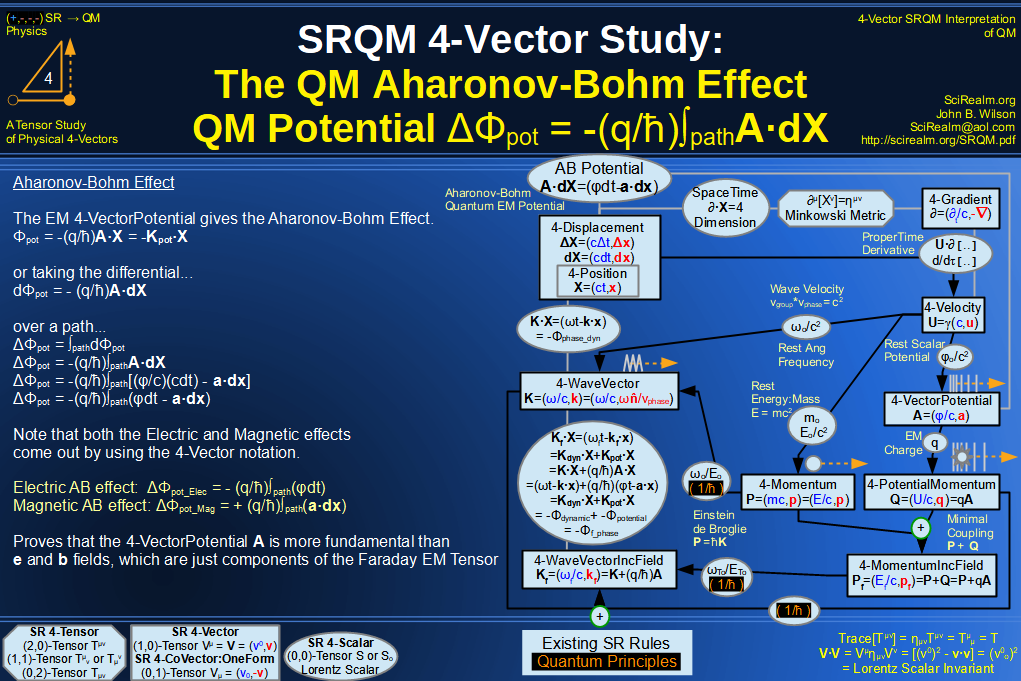SRQM 4-Vector : Four-Vector Josephson Junction Effect Diagram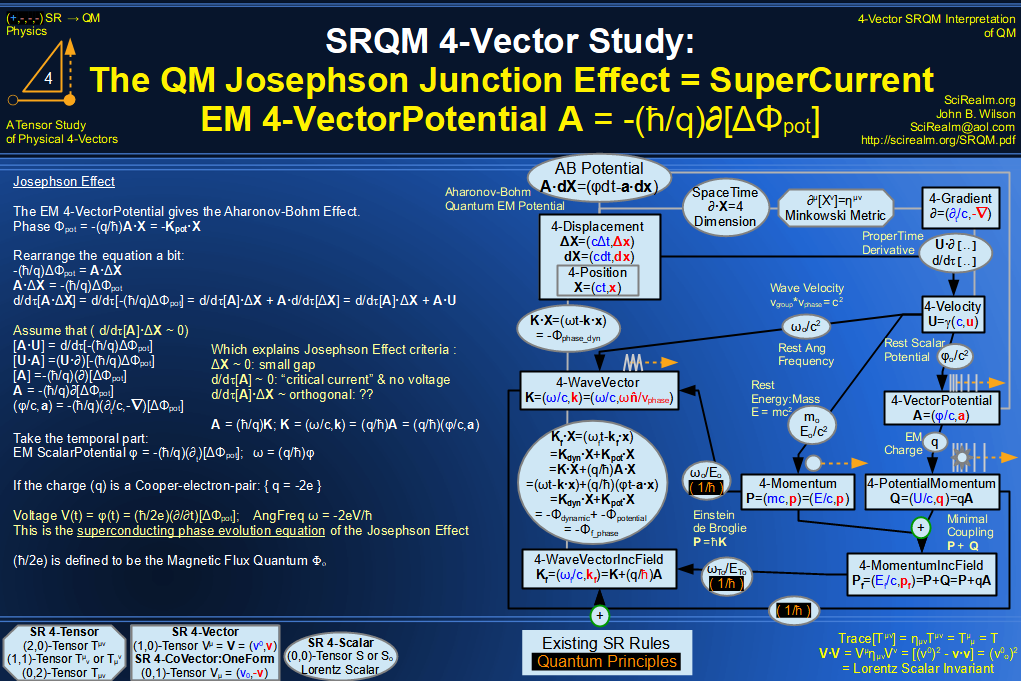SRQM 4-Vector : Four-Vector Hamilton-Jacobi vs Action, Josephson vs Aharonov-Bohm Diagram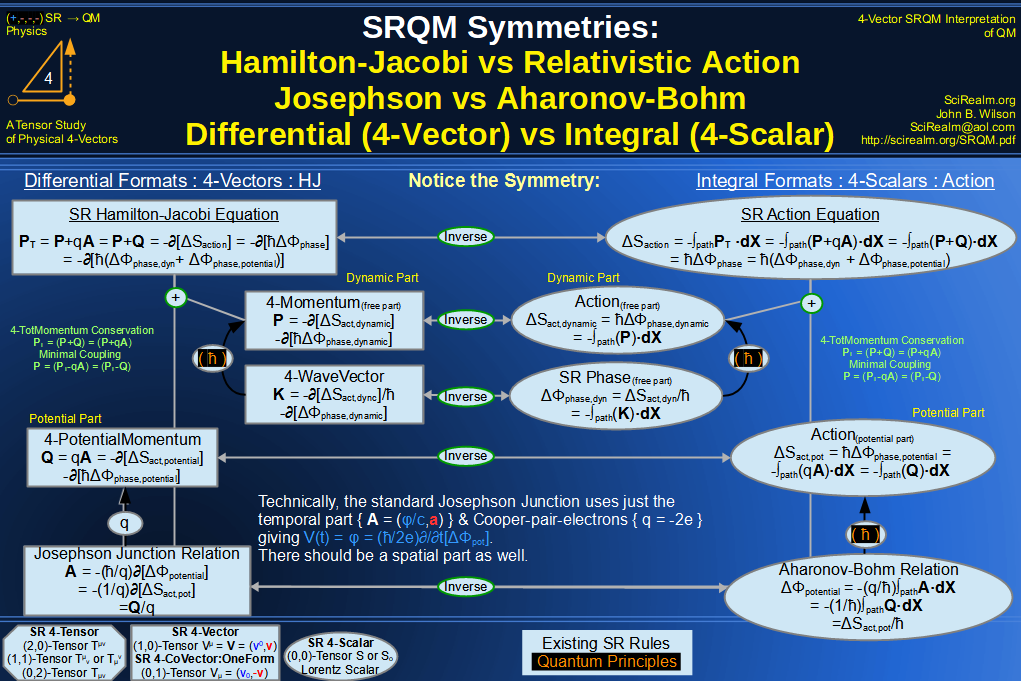SRQM 4-Vector : Four-Vector Motion of Lorentz Scalar Invariants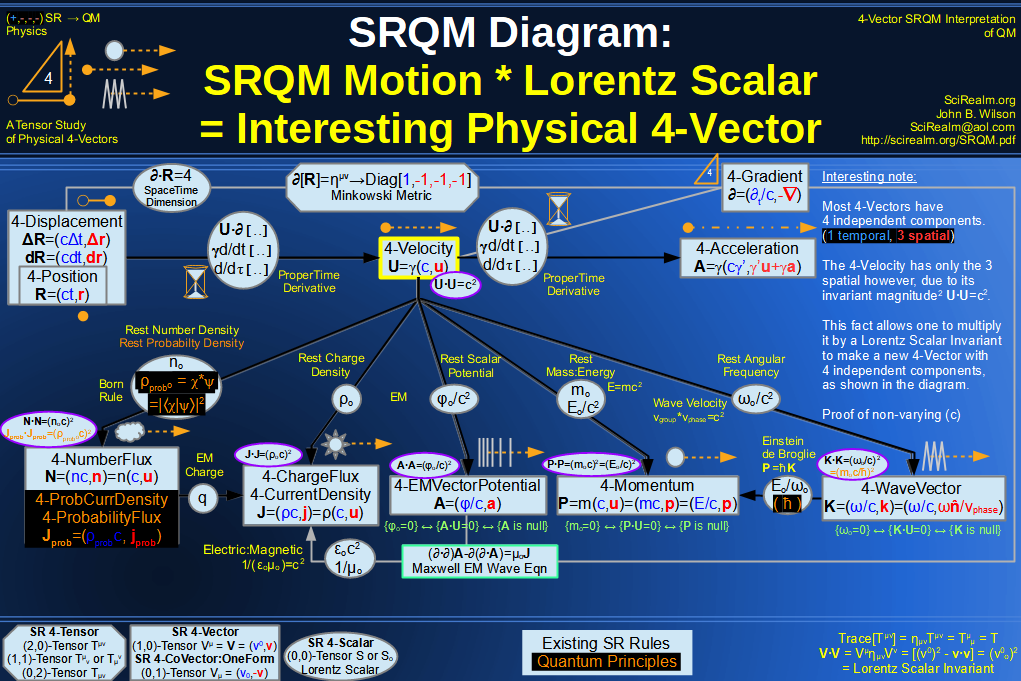SRQM 4-Vector : Four-Vector Motion of Lorentz Scalar Invariants, Conservation Laws & Continuity Equations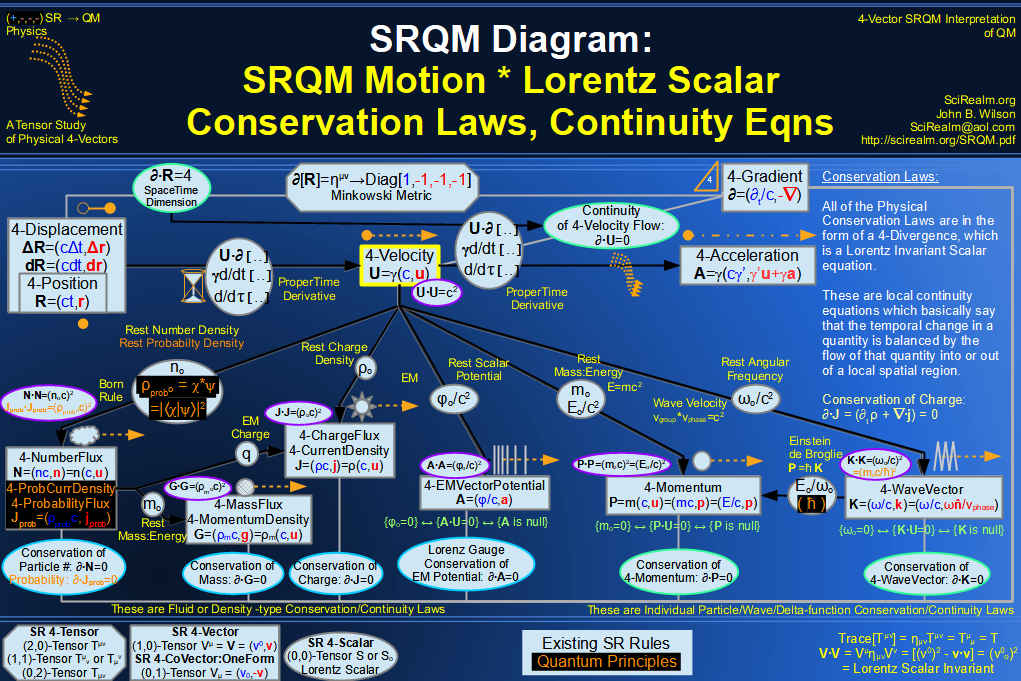SRQM 4-Vector : Four-Vector Speed of Light (c)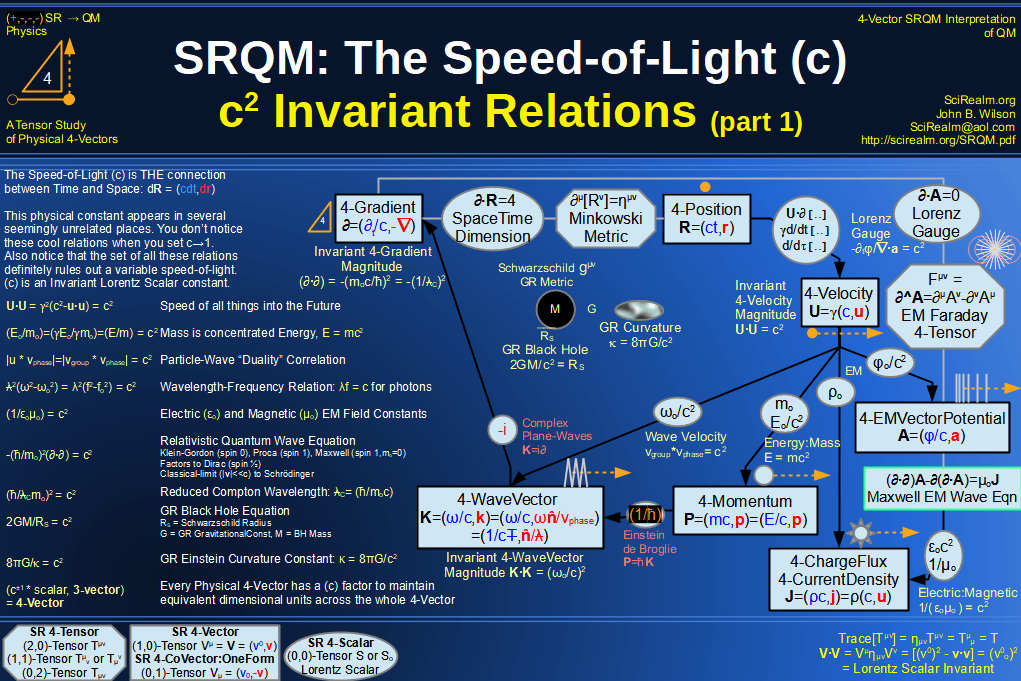SRQM 4-Vector : Four-Vector Minimal Coupling Conservation of 4-TotalMomentum)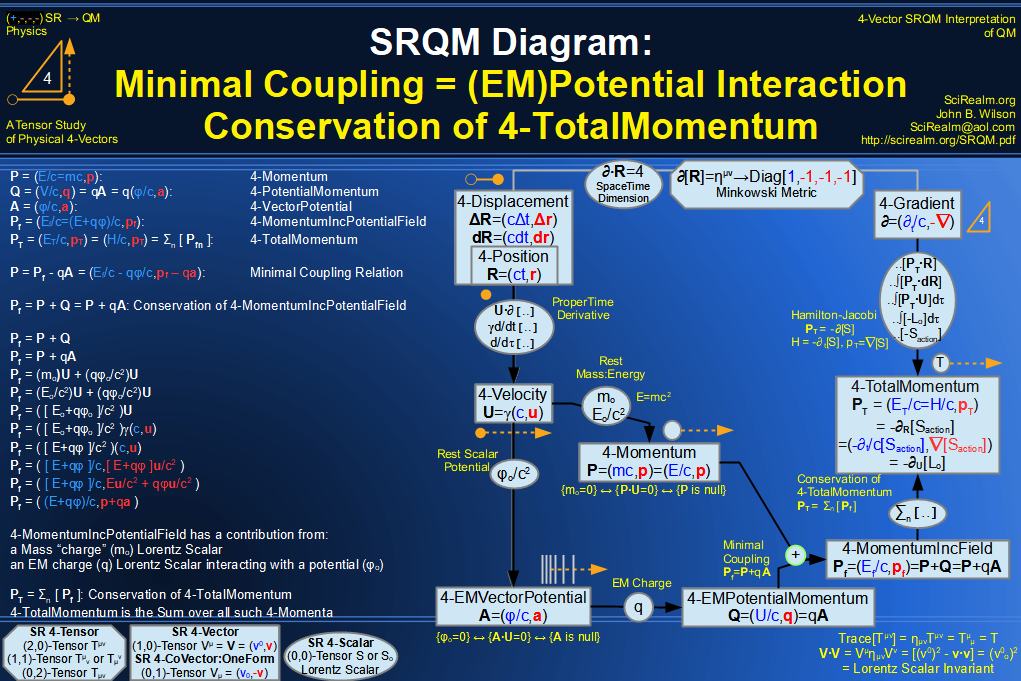SRQM 4-Vector : Four-Vector Relativistic Action (S) Diagram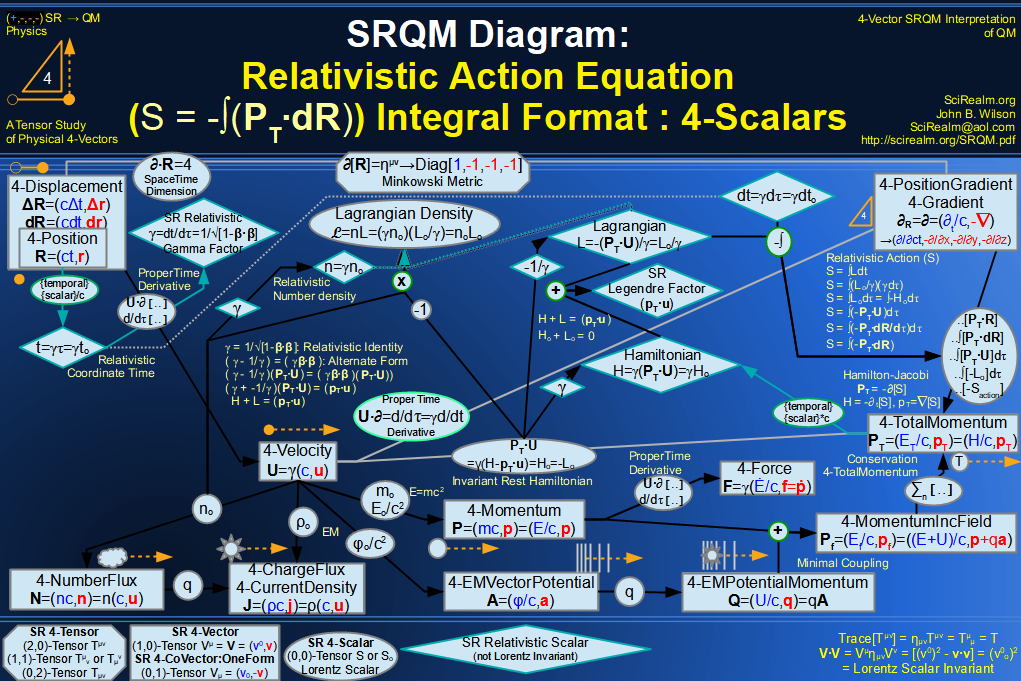SRQM 4-Vector : Four-Vector Relativistic Lagrangian Hamiltonian Diagram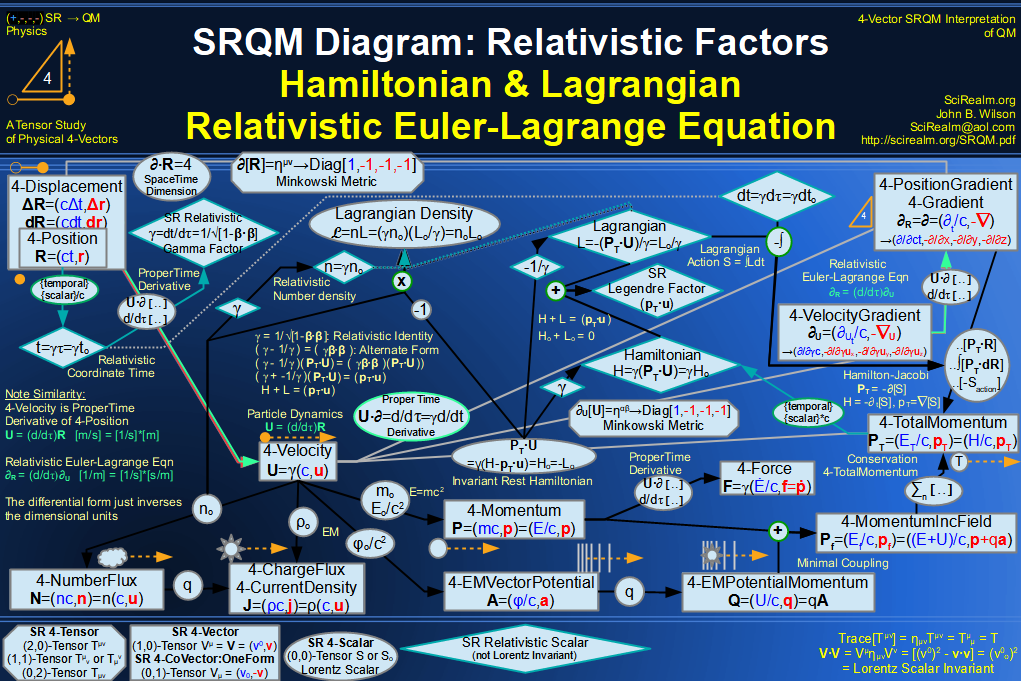SRQM 4-Vector : Four-Vector Relativistic Euler-Lagrange Equation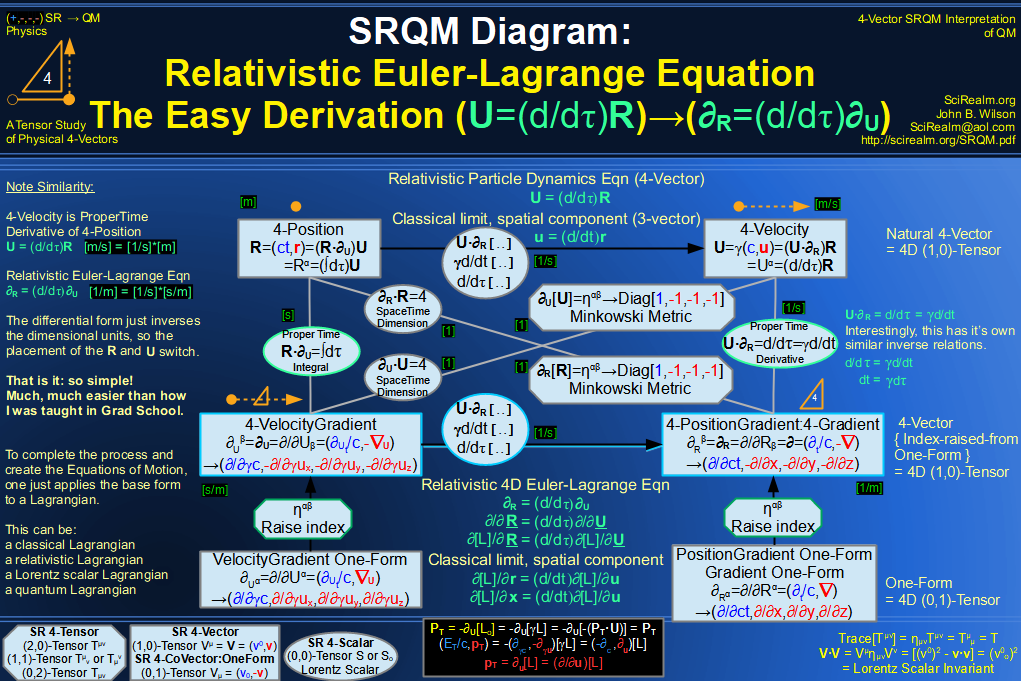SRQM 4-Vector : Four-Vector Relativistic EM Equations of Motion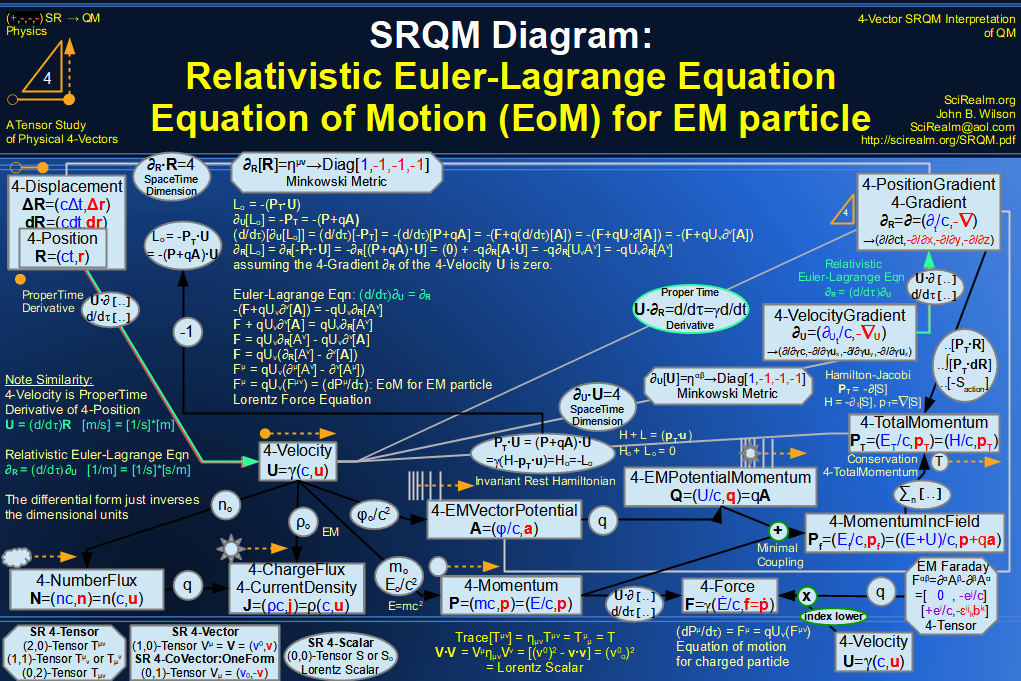SRQM 4-Vector : Four-Vector Einstein-de Broglie Relation hbar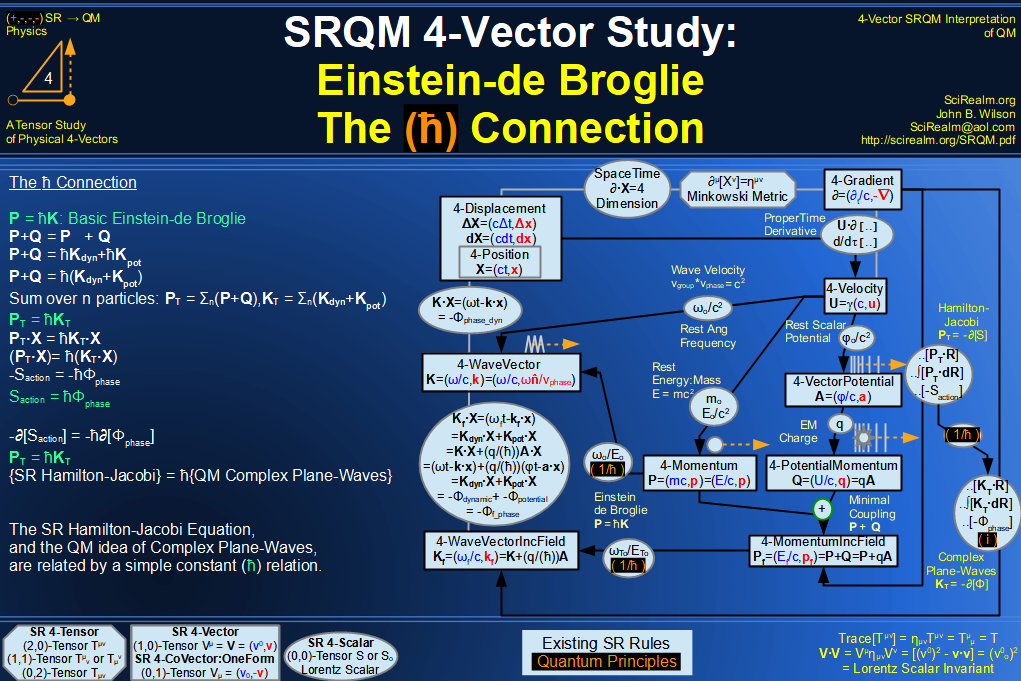SRQM 4-Vector : Four-Vector Quantum Canonical Commutation Relation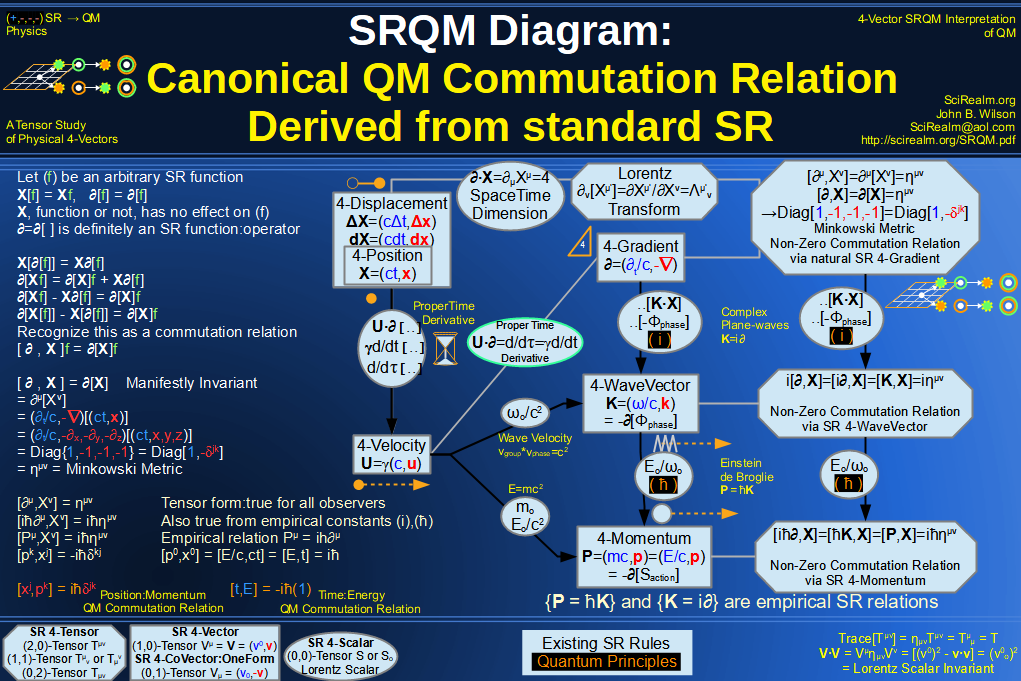SRQM 4-Vector : Four-Vector QM Schroedinger Relation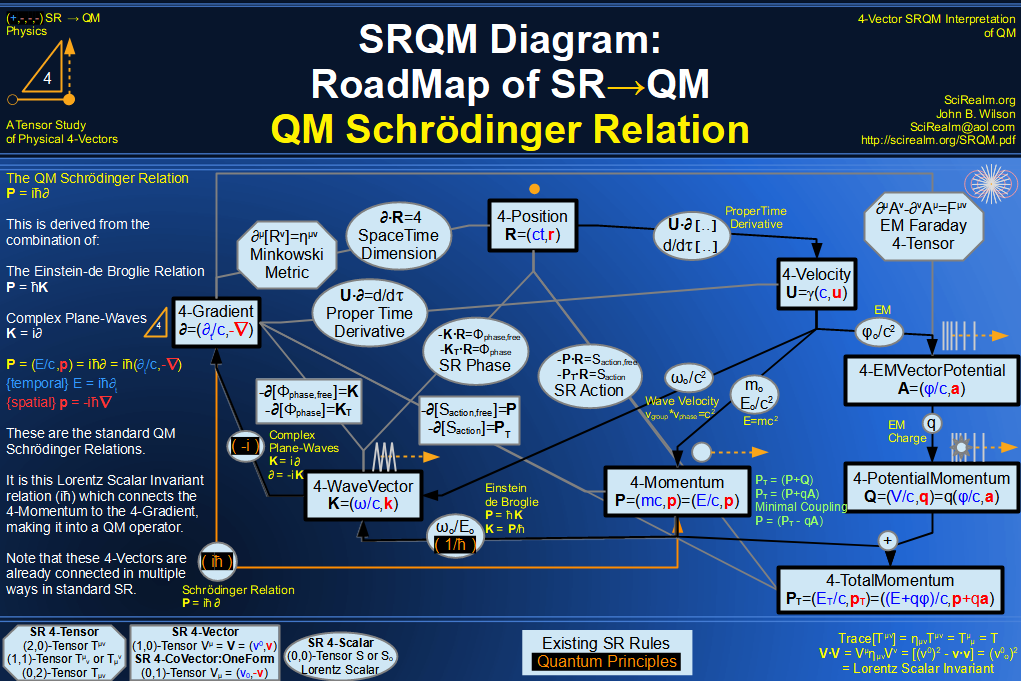SRQM 4-Vector : Four-Vector Quantum Probability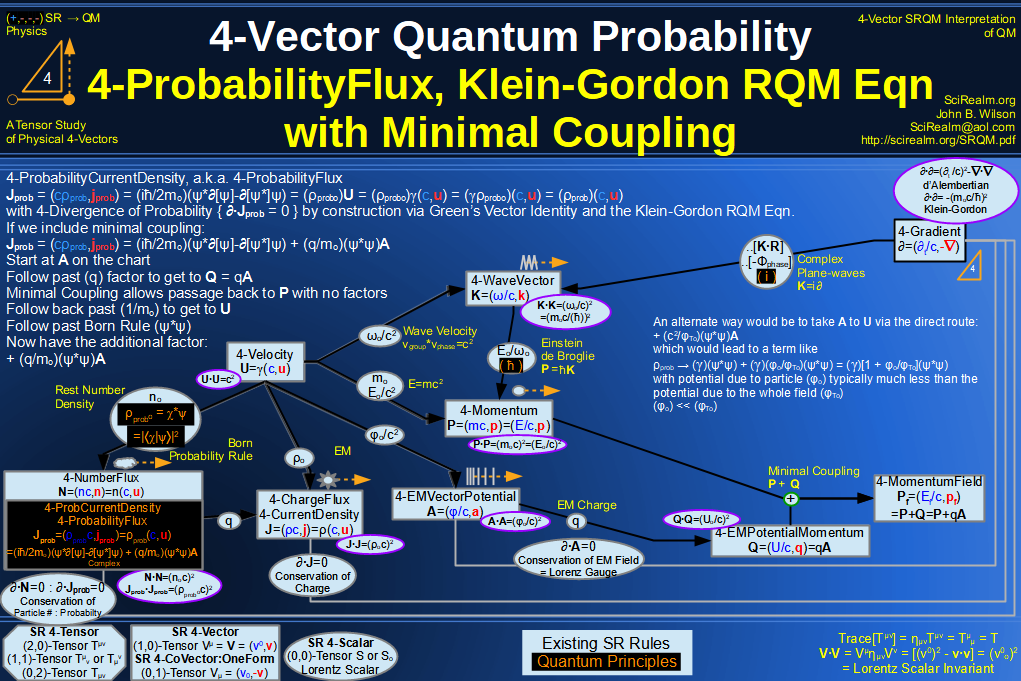SRQM 4-Vector : Four-Vector CPT Theorem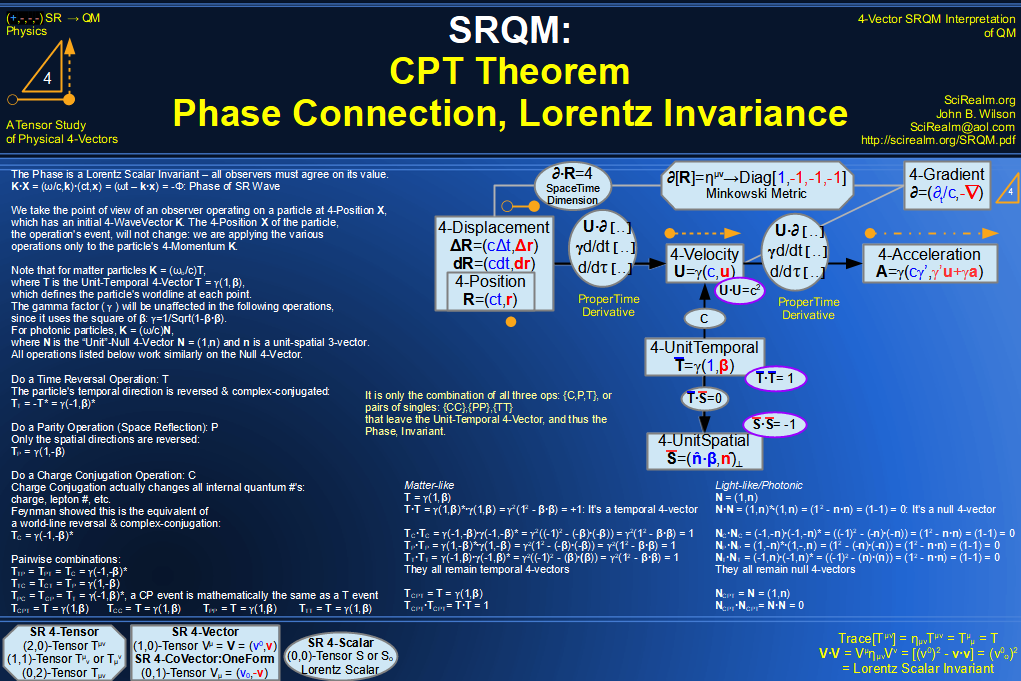SRQM 4-Vector : Four-Vector Lorentz Transforms Connection Map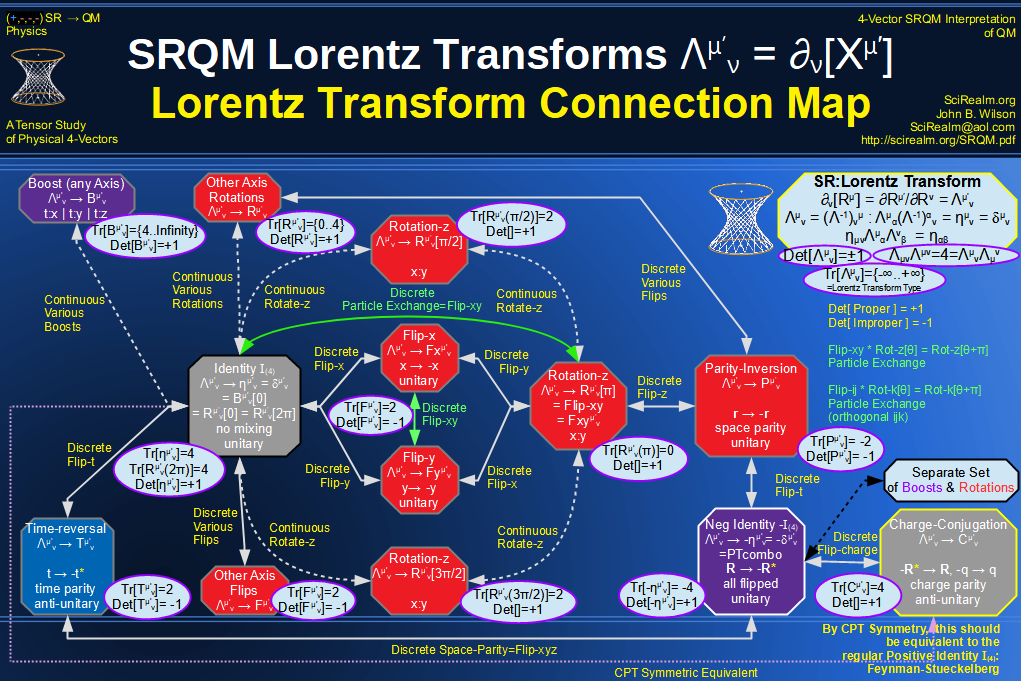SRQM 4-Vector : Four-Vector Lorentz Discrete Transforms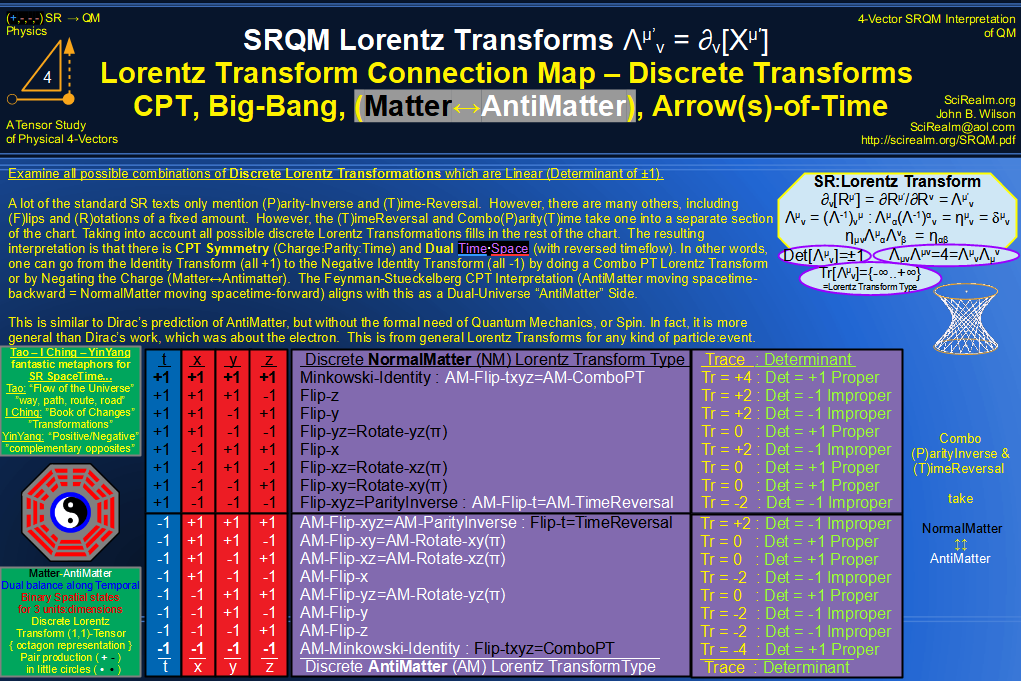SRQM 4-Vector : Four-Vector Lorentz Transforms - Trace Identification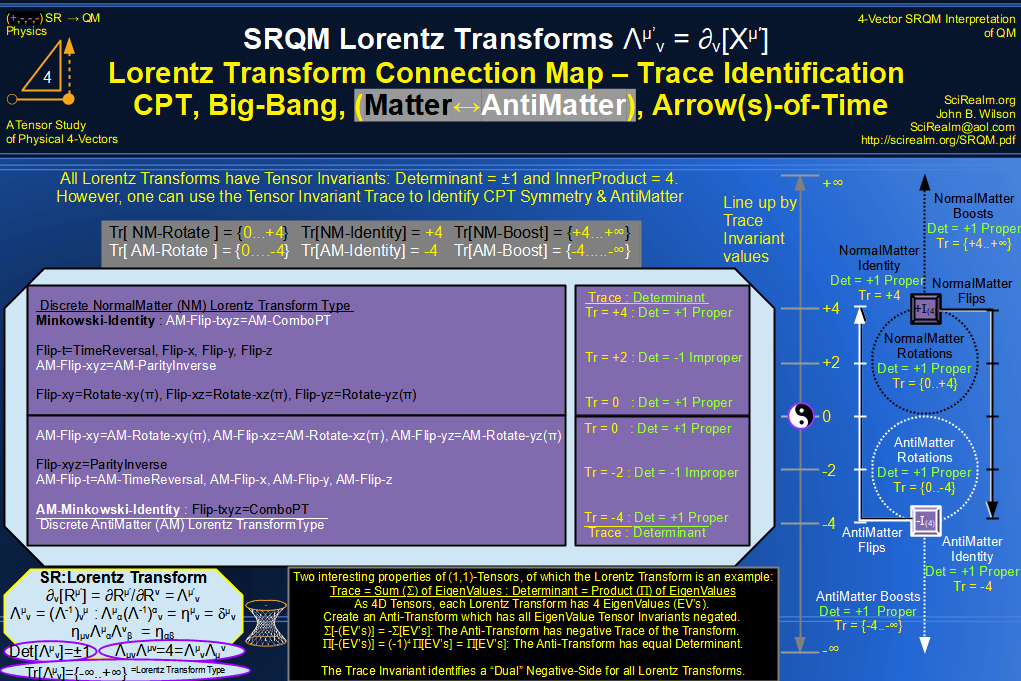SRQM 4-Vector : Four-Vector Lorentz Lorentz Transforms-Interpretations
CPT Symmetry, Baryon Asymmetry Problem Solution, Matter-Antimatter Symmetry Solution, Arrow-of-Time Problem Solution, Big-Bang!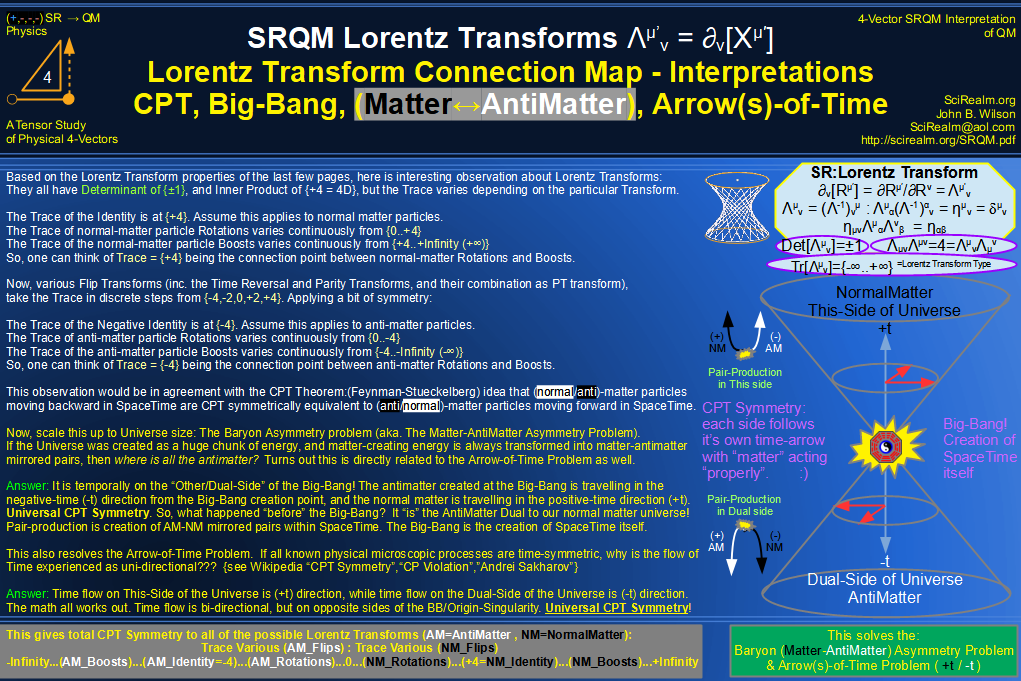See SRQM: QM from SR - The 4-Vector RoadMap (.html)
See SRQM: QM from SR - The 4-Vector RoadMap (.pdf)The Science Realm: John's Virtual Sci-Tech Universe John's Science & Math Stuff: | About John | Send email to John 4-Vectors | Ambigrams | Antipodes | Covert Ops Fragments | Cyrillic Projector | Forced Induction (Sums Of Powers Of Integers) | Fractals | Frontiers | JavaScript Graphics | Kid Science | Kryptos | Photography | Prime Sieve | QM from SR | QM from SR-Simple RoadMap | Quantum Phase | Quotes | RuneQuest Cipher Challenge | Scientific Calculator | Secret Codes & Ciphers | Science+Math | Sci-Pan Pantheist Poems | Stereograms | Turkish Grammar | Quantum Mechanics is derivable from Special Relativity See QM from SR-Simple RoadMap